# MSBSHSE Solutions For Class 9 Maths Part 1 Chapter 2 Real Numbers

MSBSHSE Solutions For Class 9 Maths Part 1 Chapter 2 Real Numbers consists of accurate solutions, which help the students to quickly complete their homework and prepare well for the exams. These solutions provide students an advantage with practical questions. Each step in the solution is explained to match students’ understanding. To score good marks in Class 9 Mathematics examination, it is advised they solve questions provided at the end of each chapter in the Maharashtra Board Textbooks for Class 9. In the Maharashtra State Board Solutions for Class 9 Chapter 2, students will learn and solve problems based on topics like properties of rational numbers, properties of irrational numbers, surds, comparison of quadratic surds, operations on quadratic surds and Rationalization of quadratic surds.

## Download the PDF of Maharashtra Solutions For Class 9 Maths Part 1 Chapter 2 Real Numbers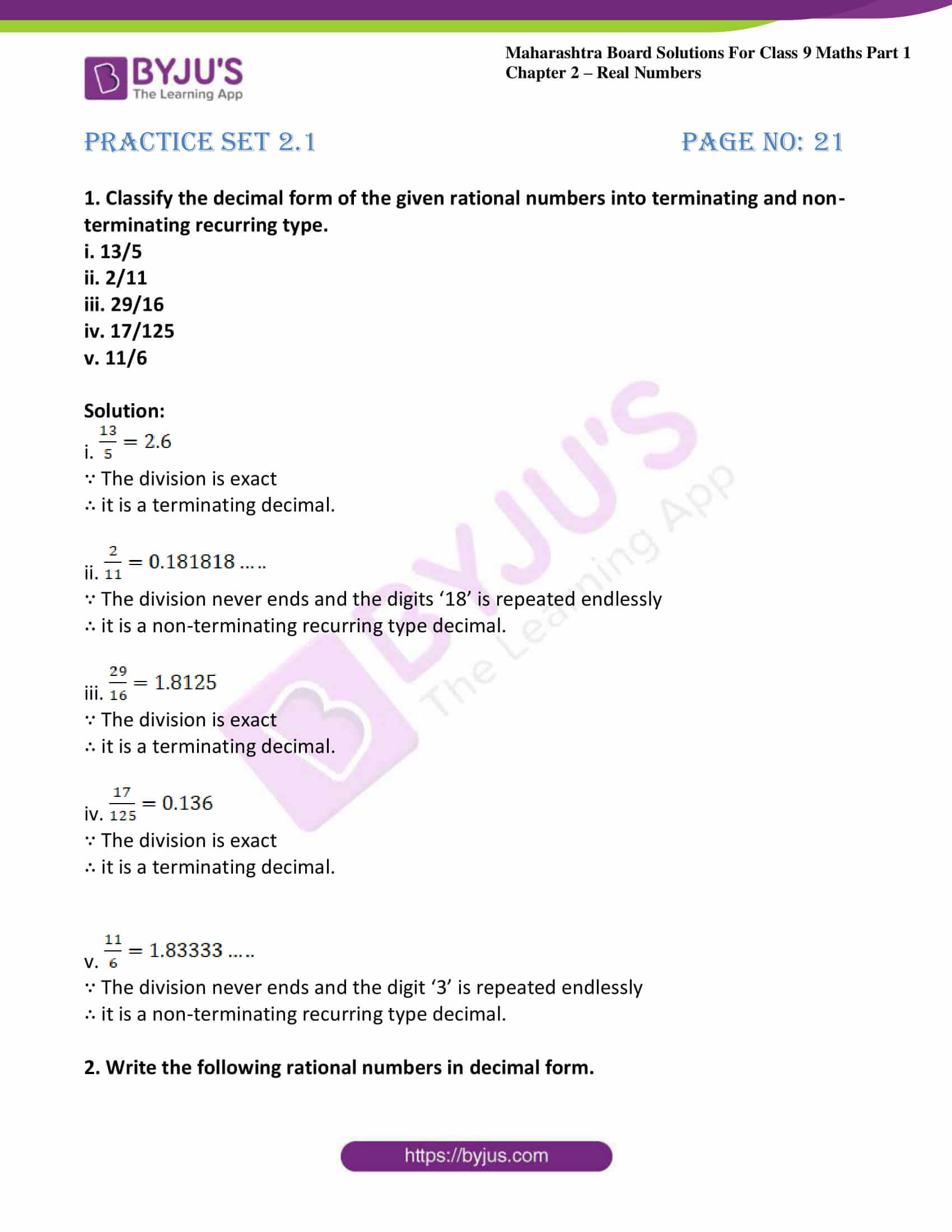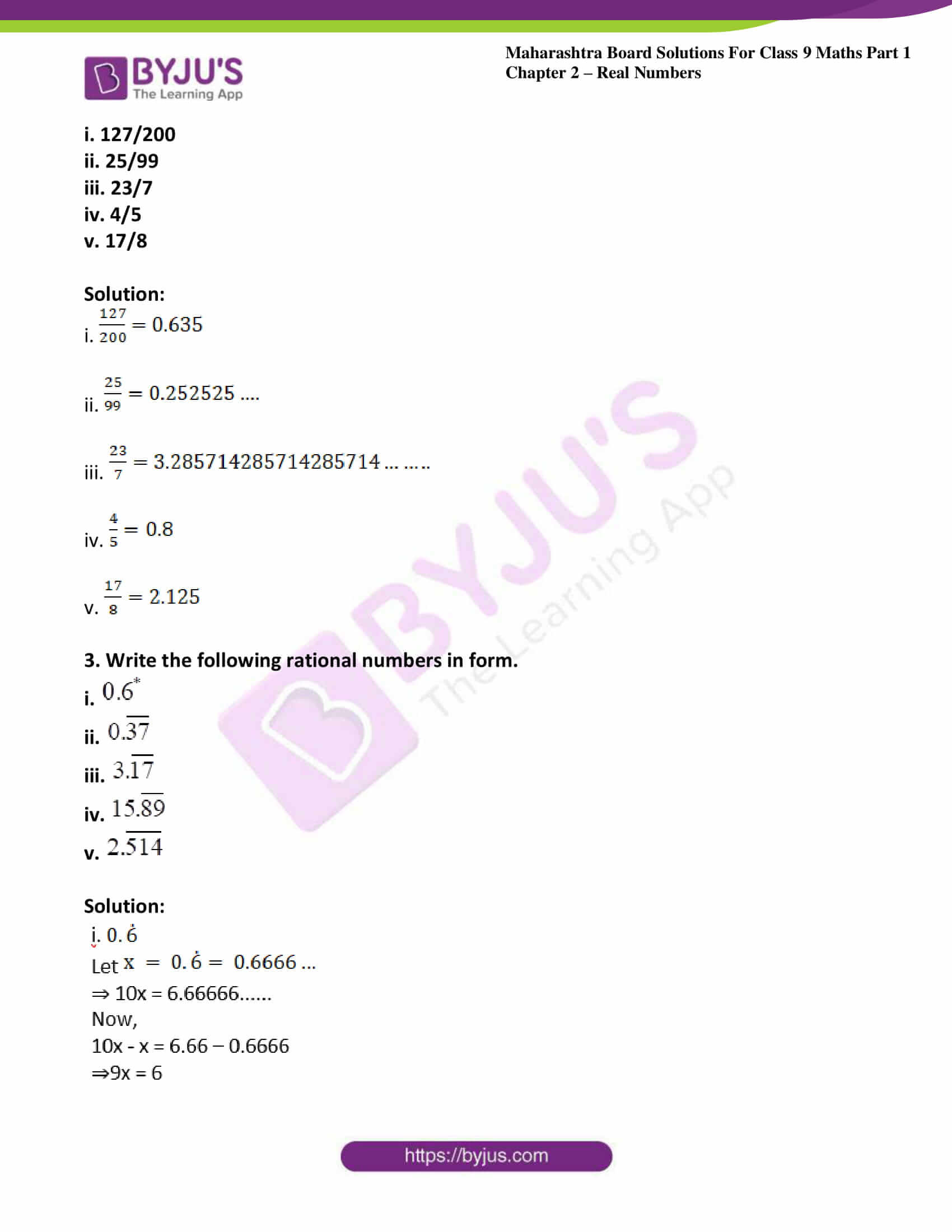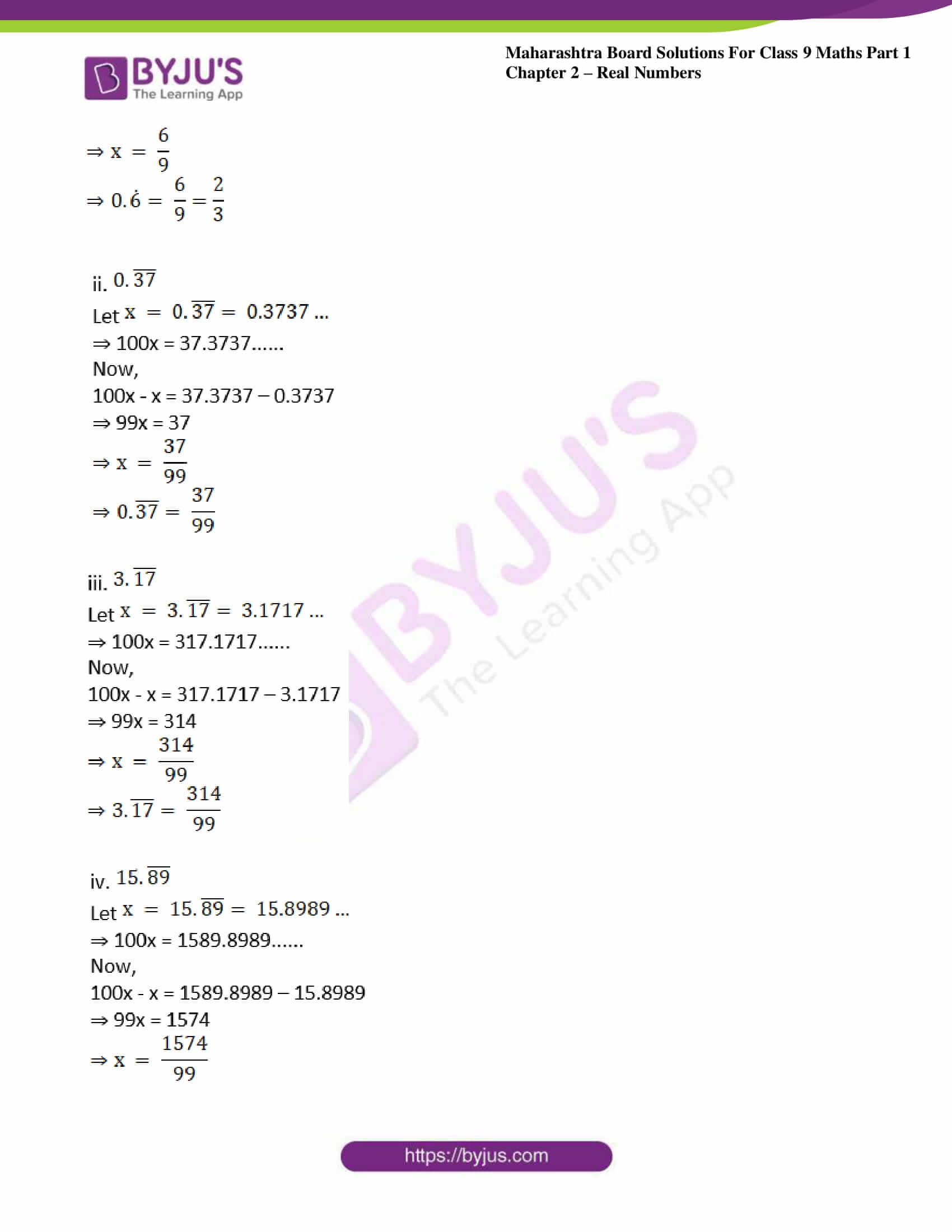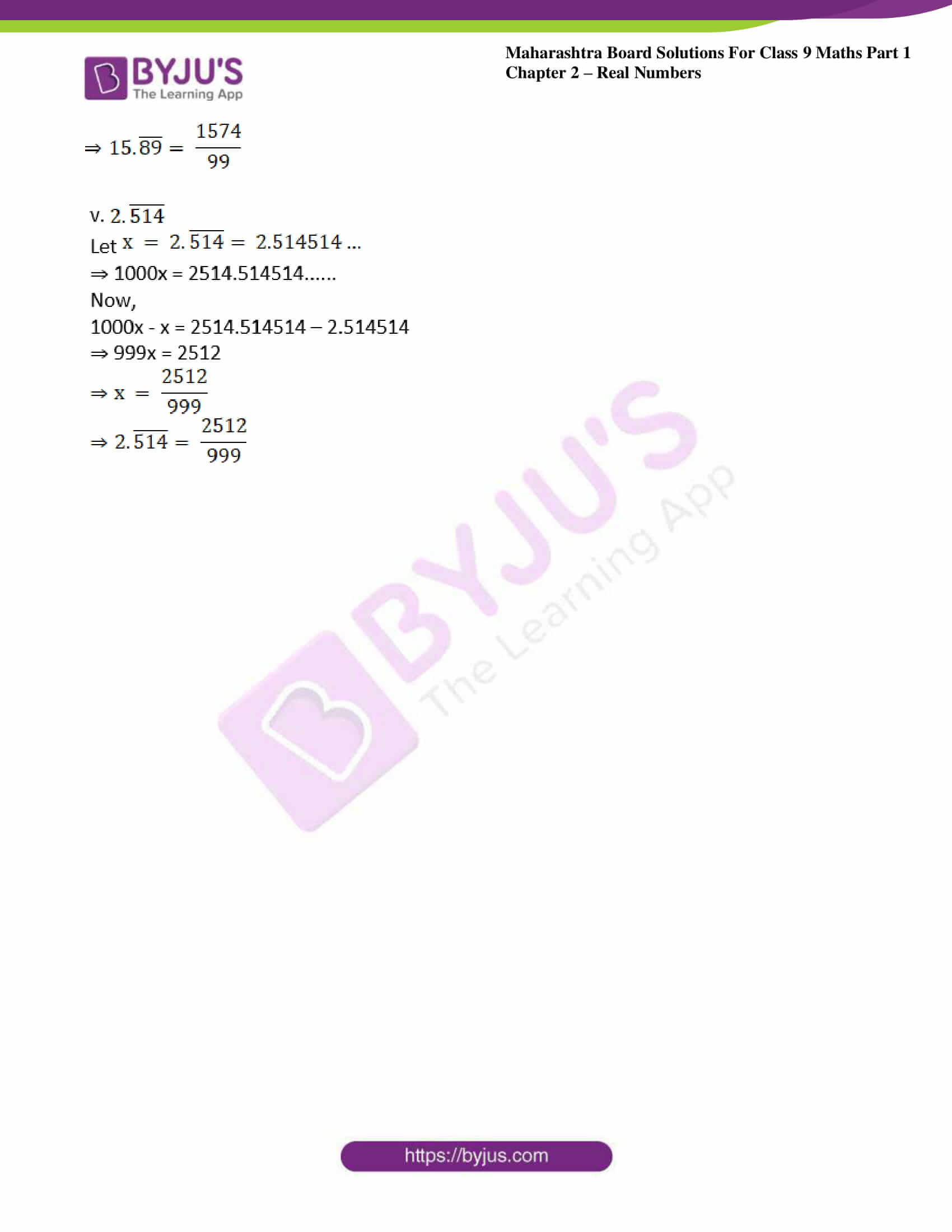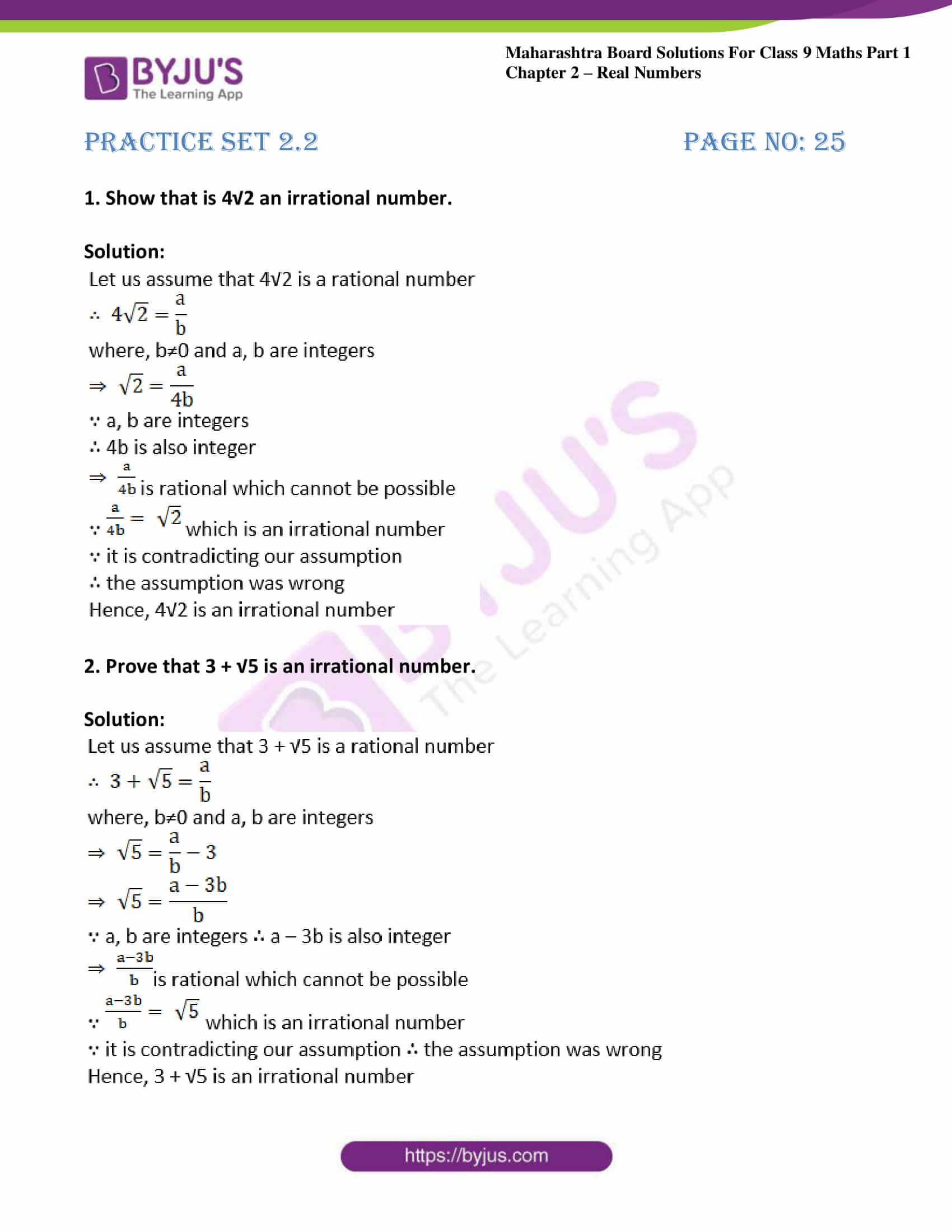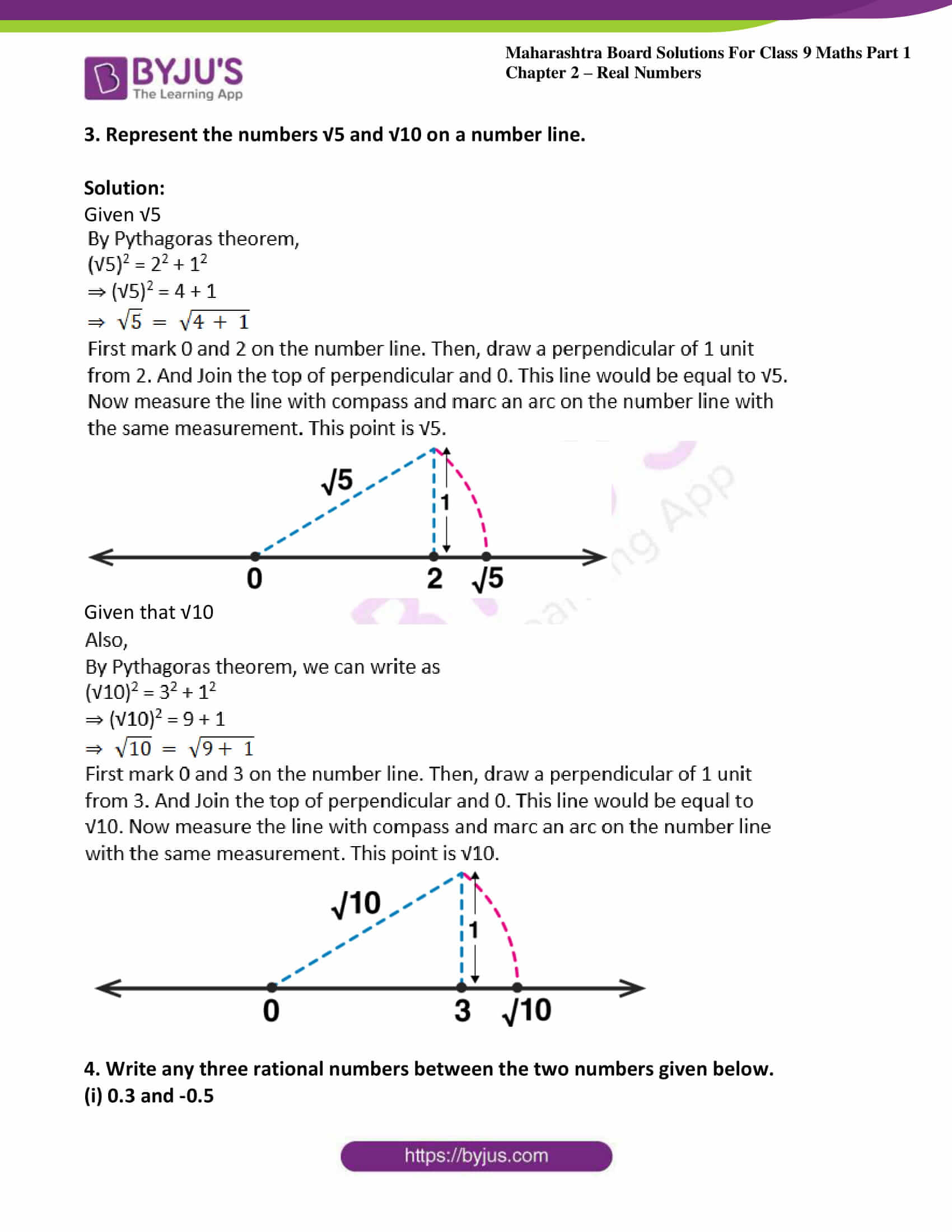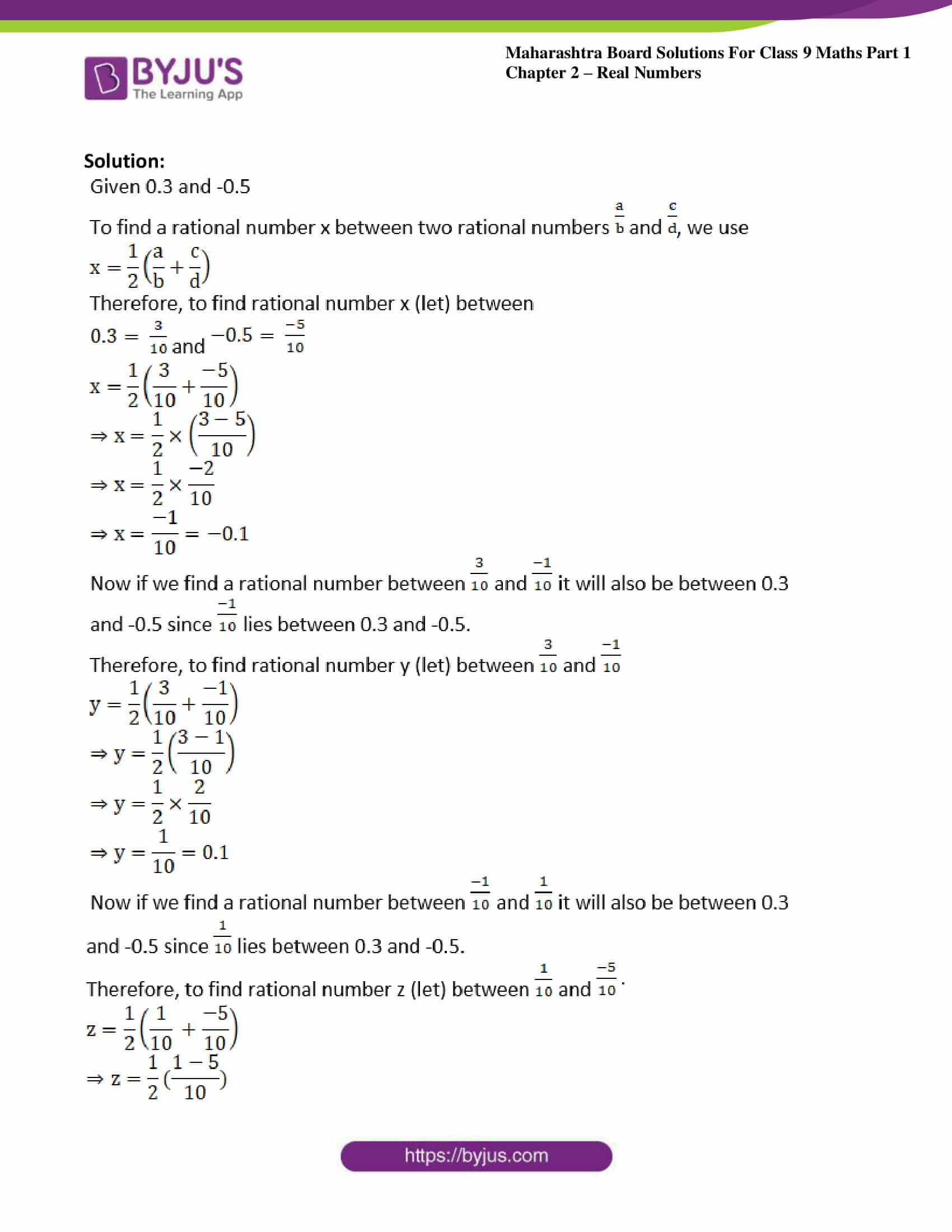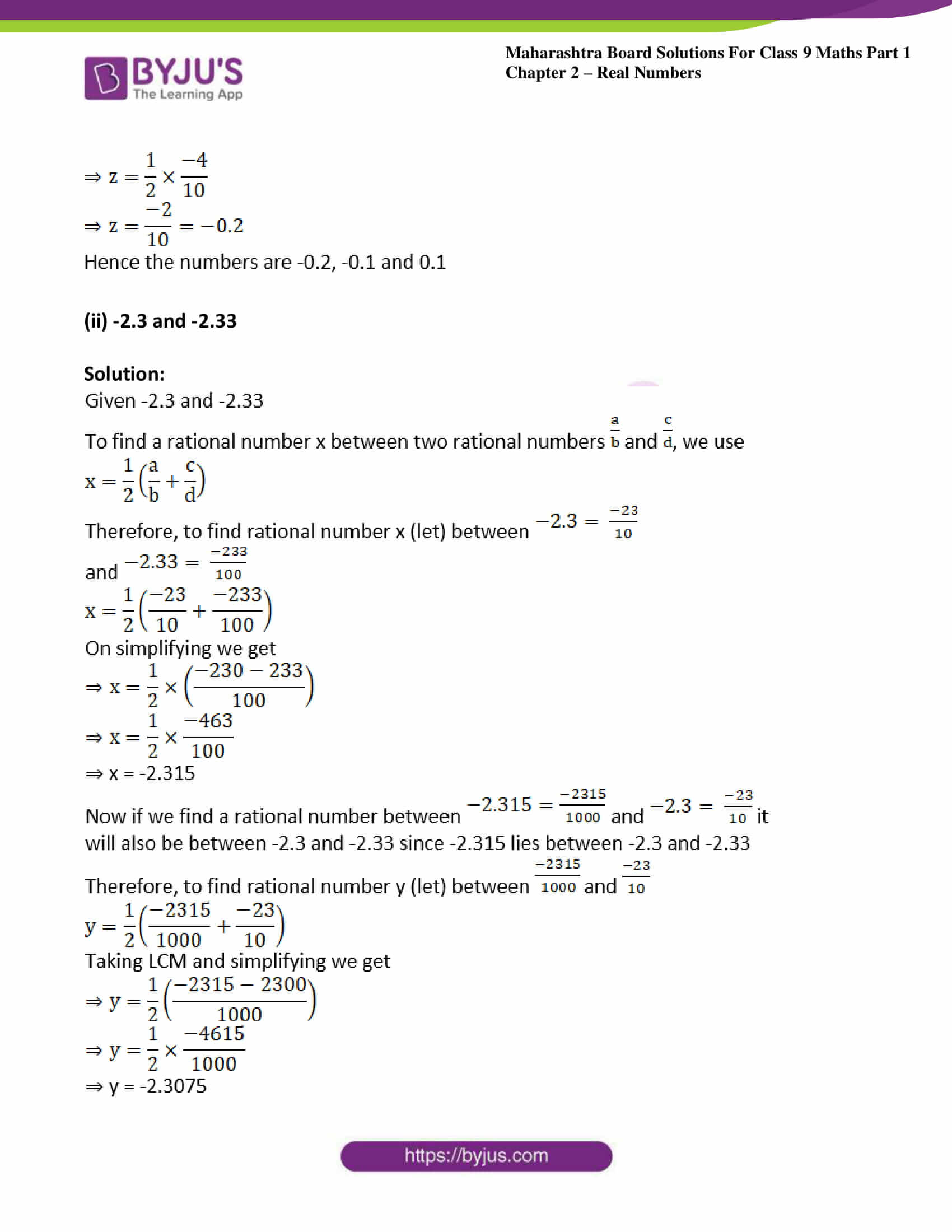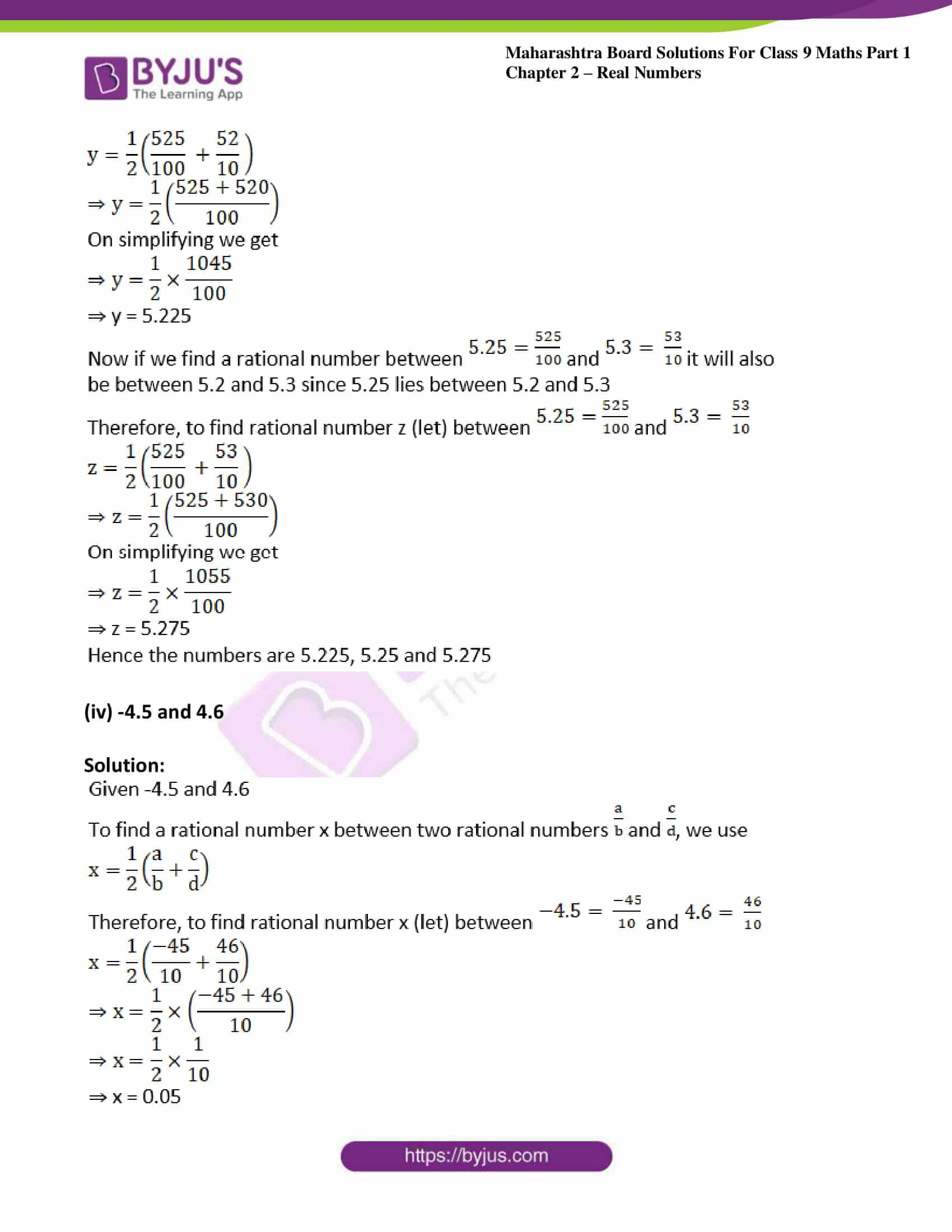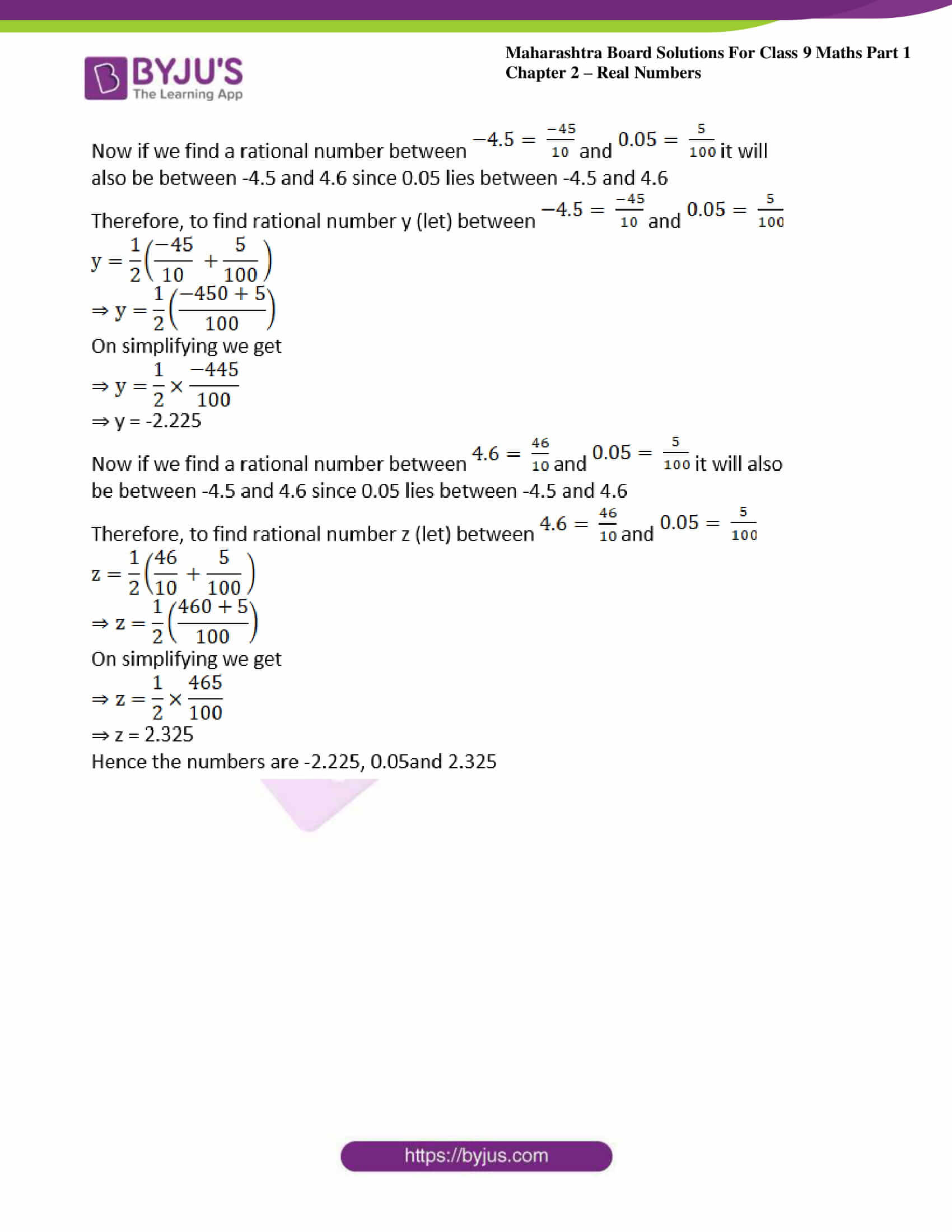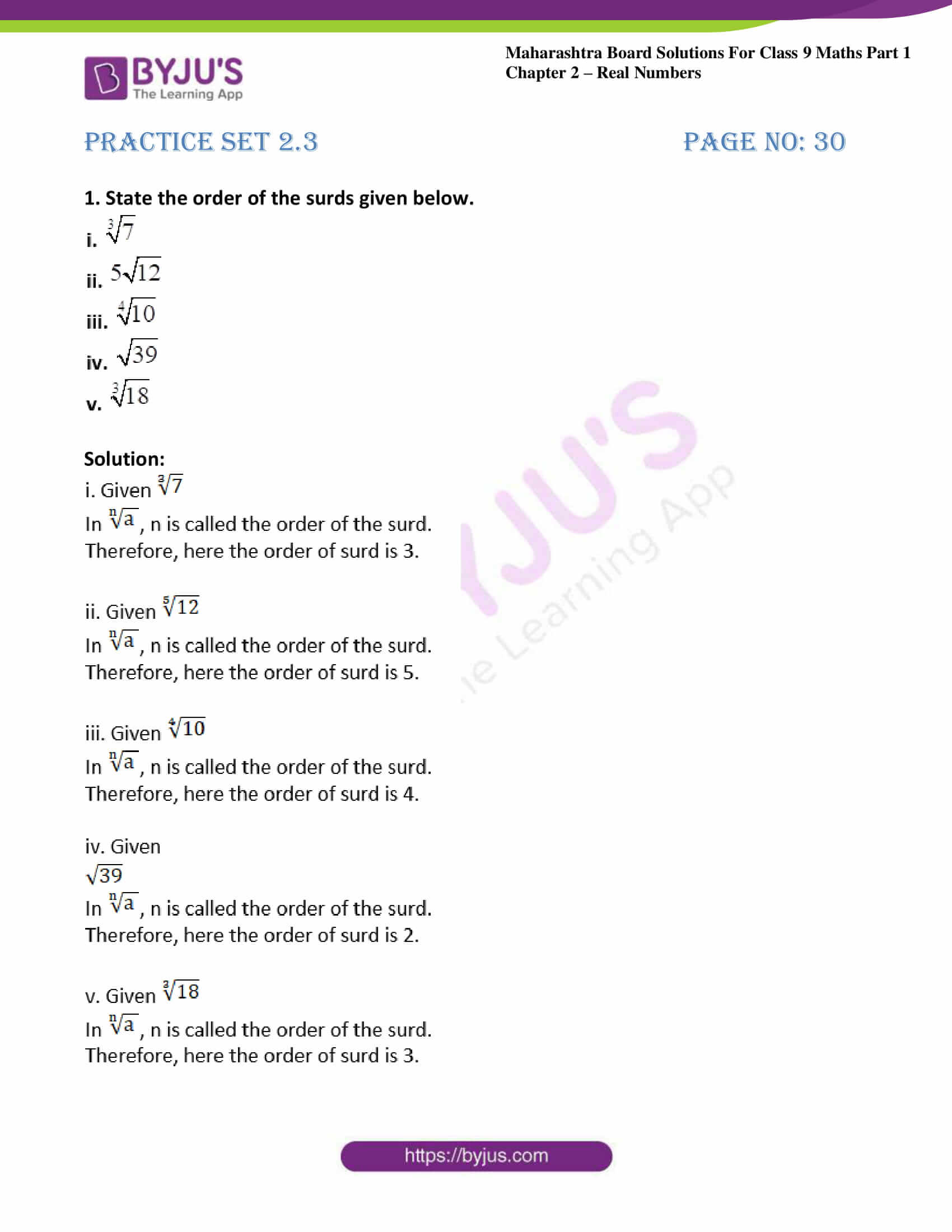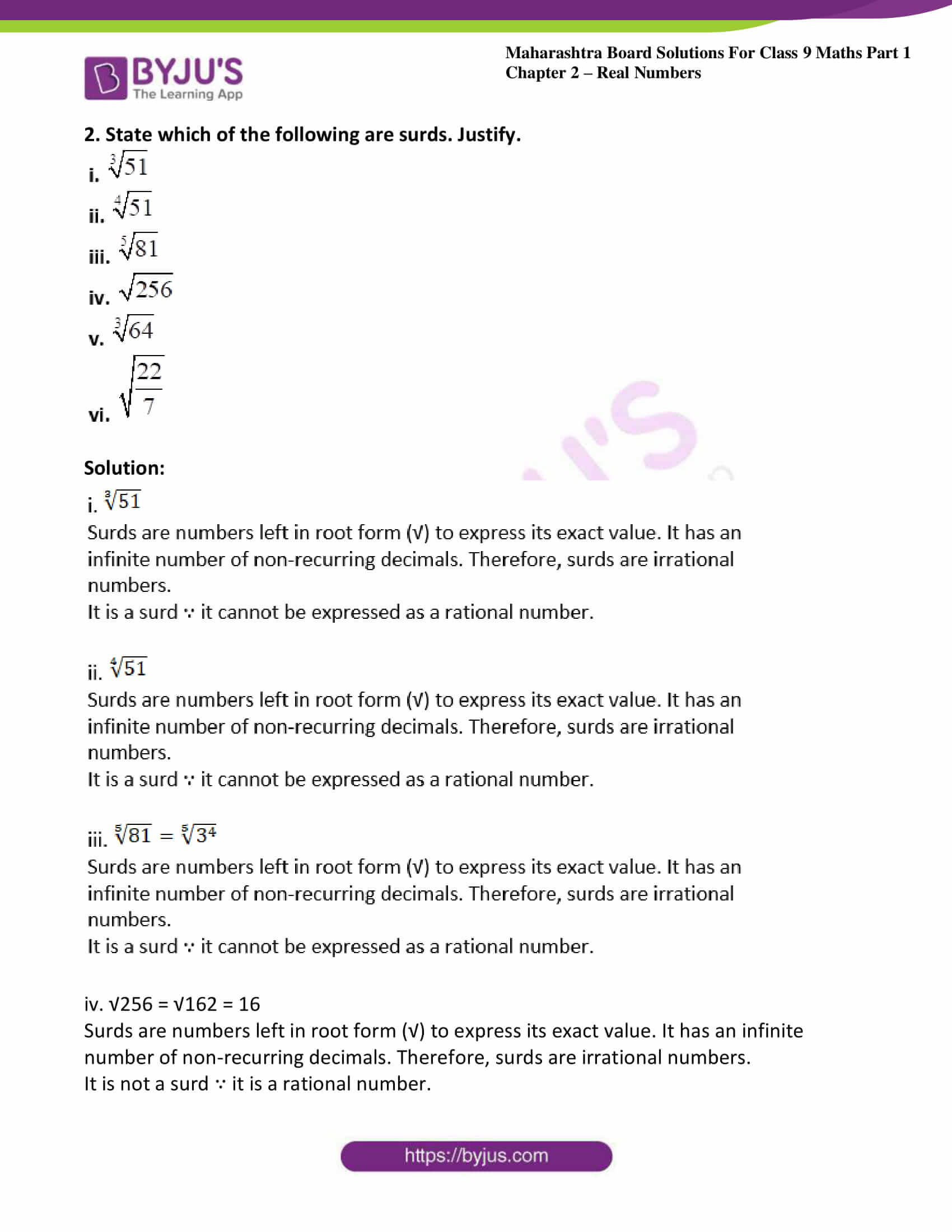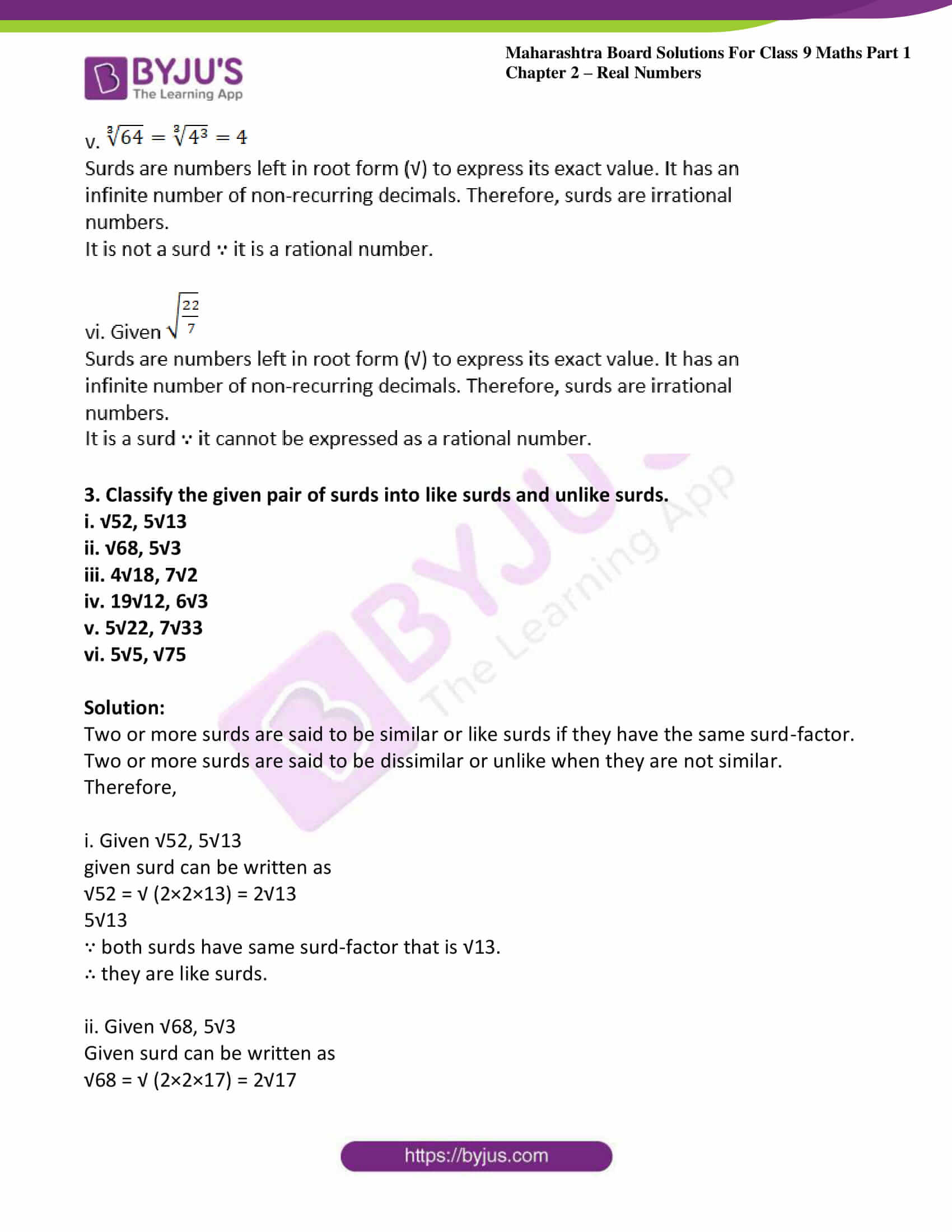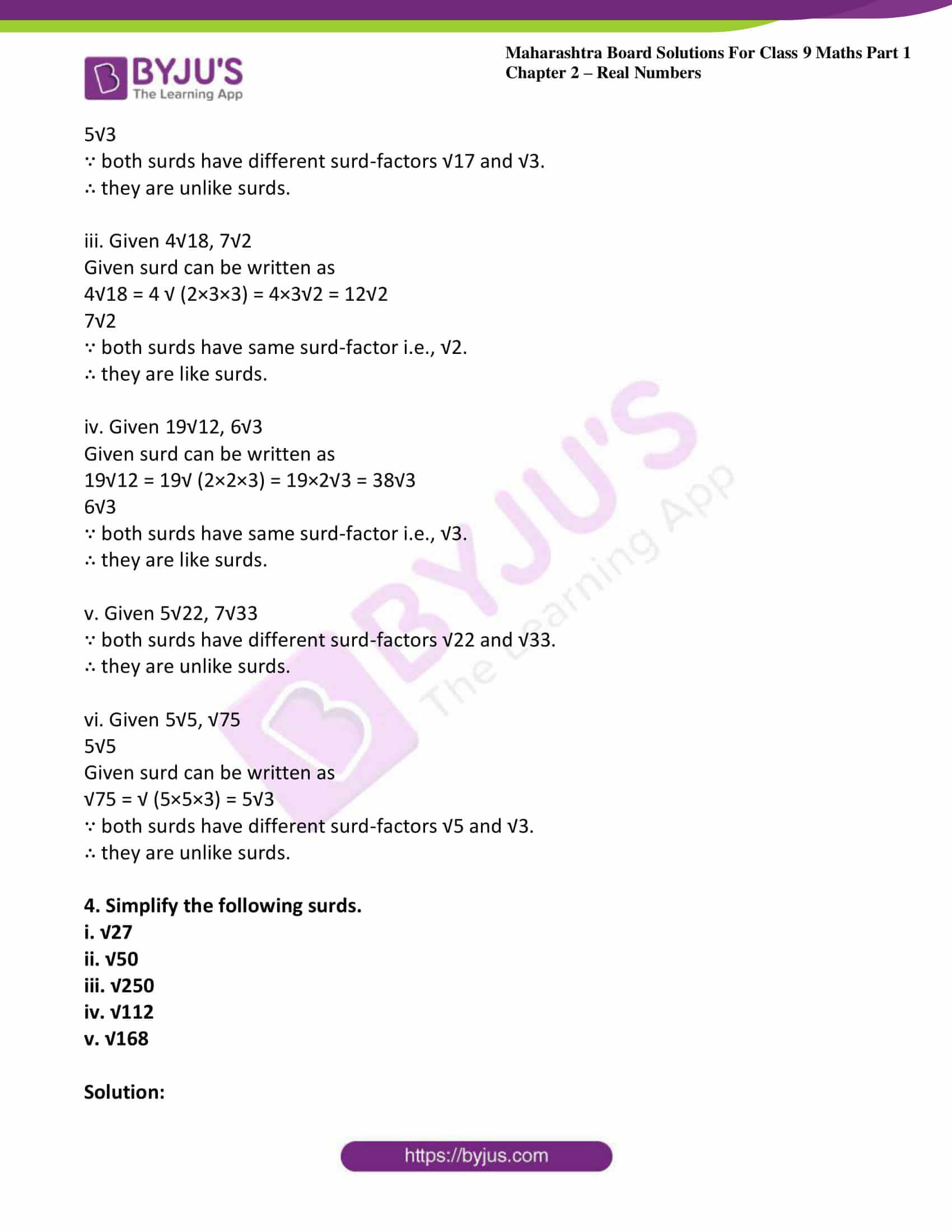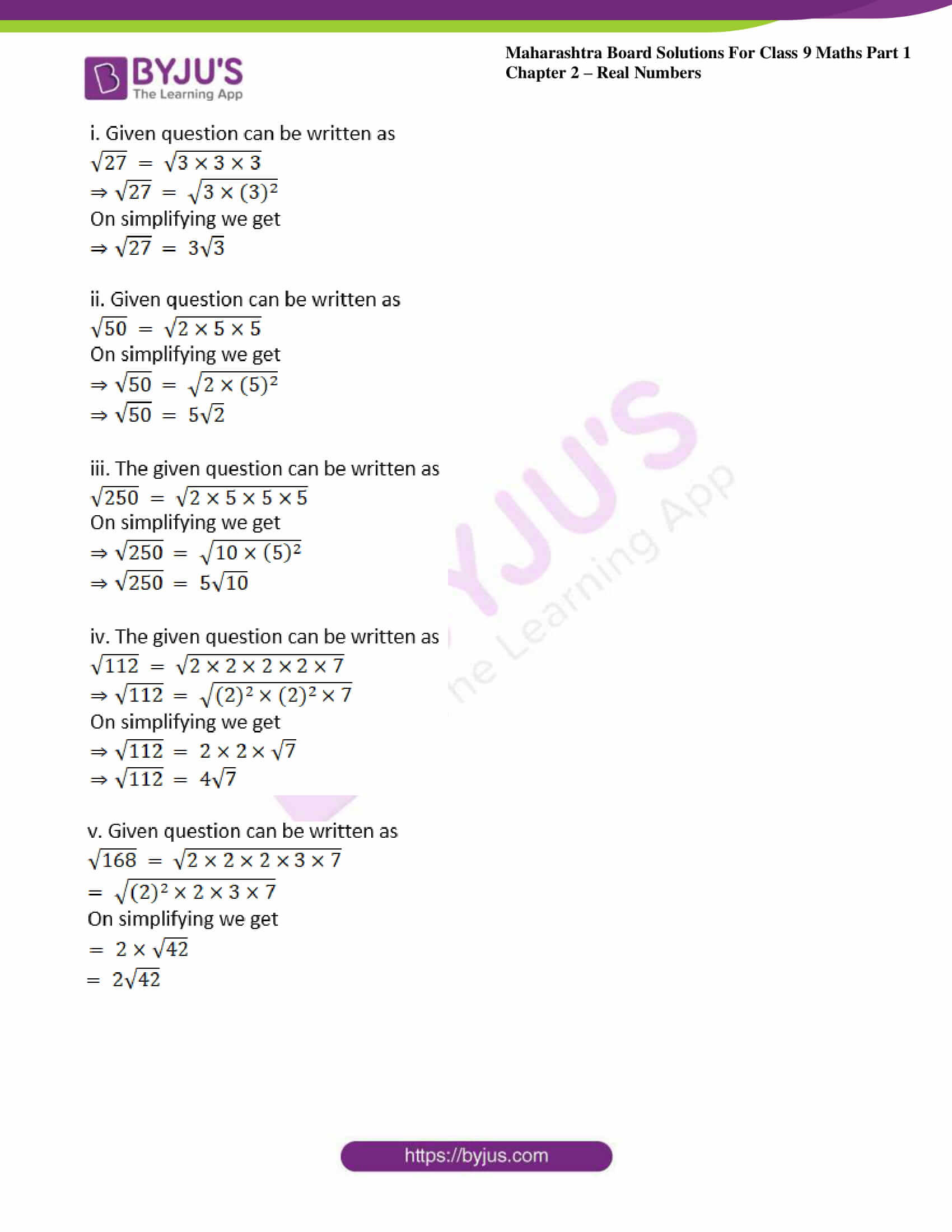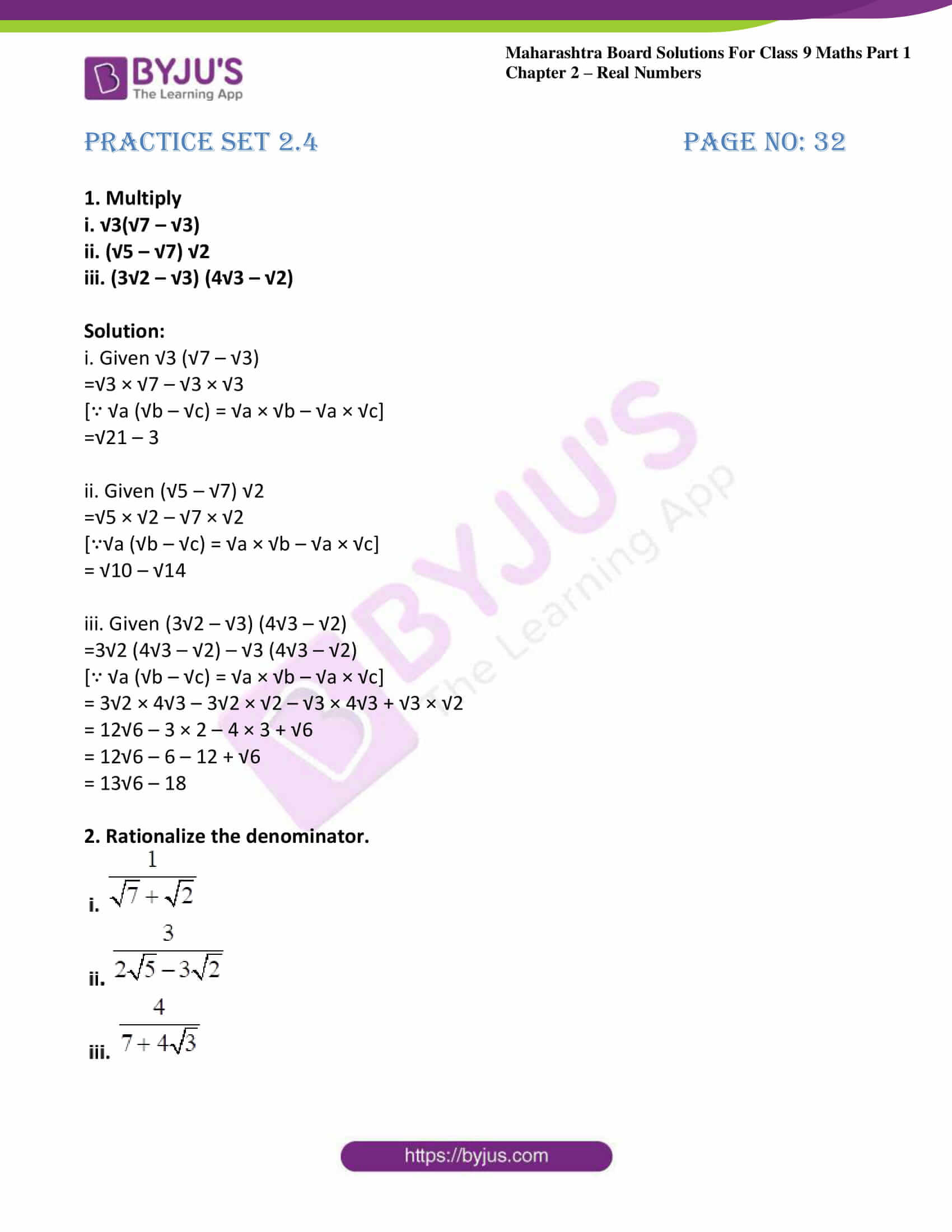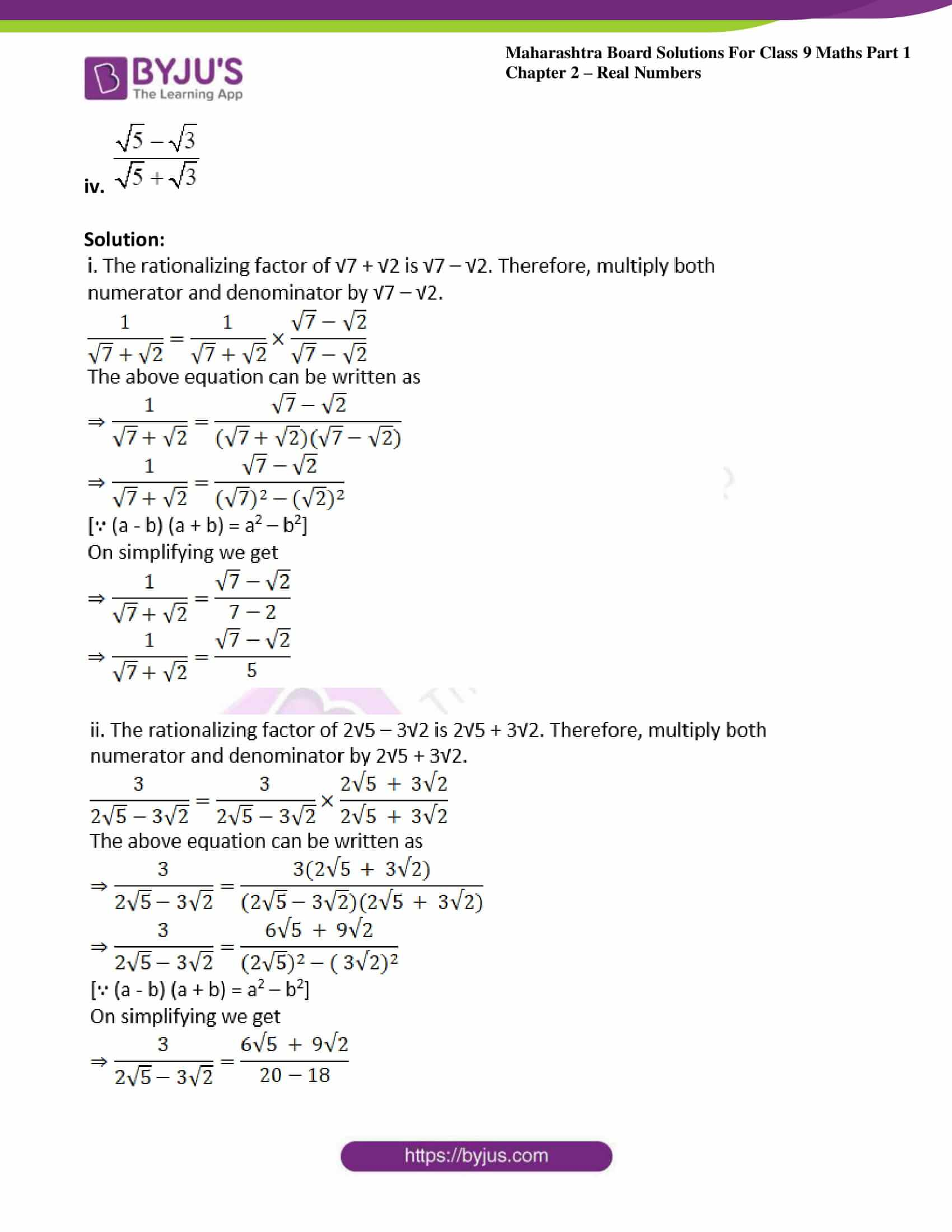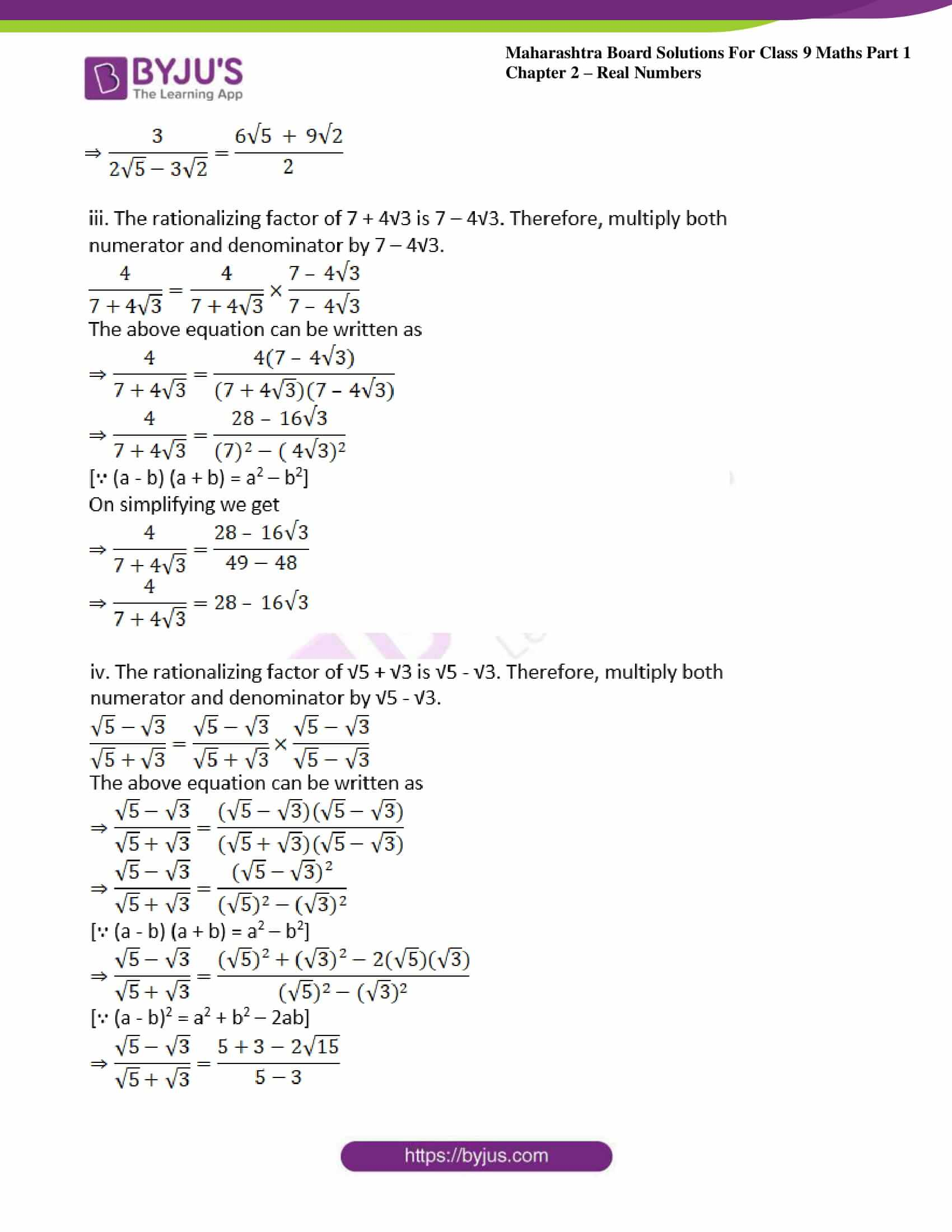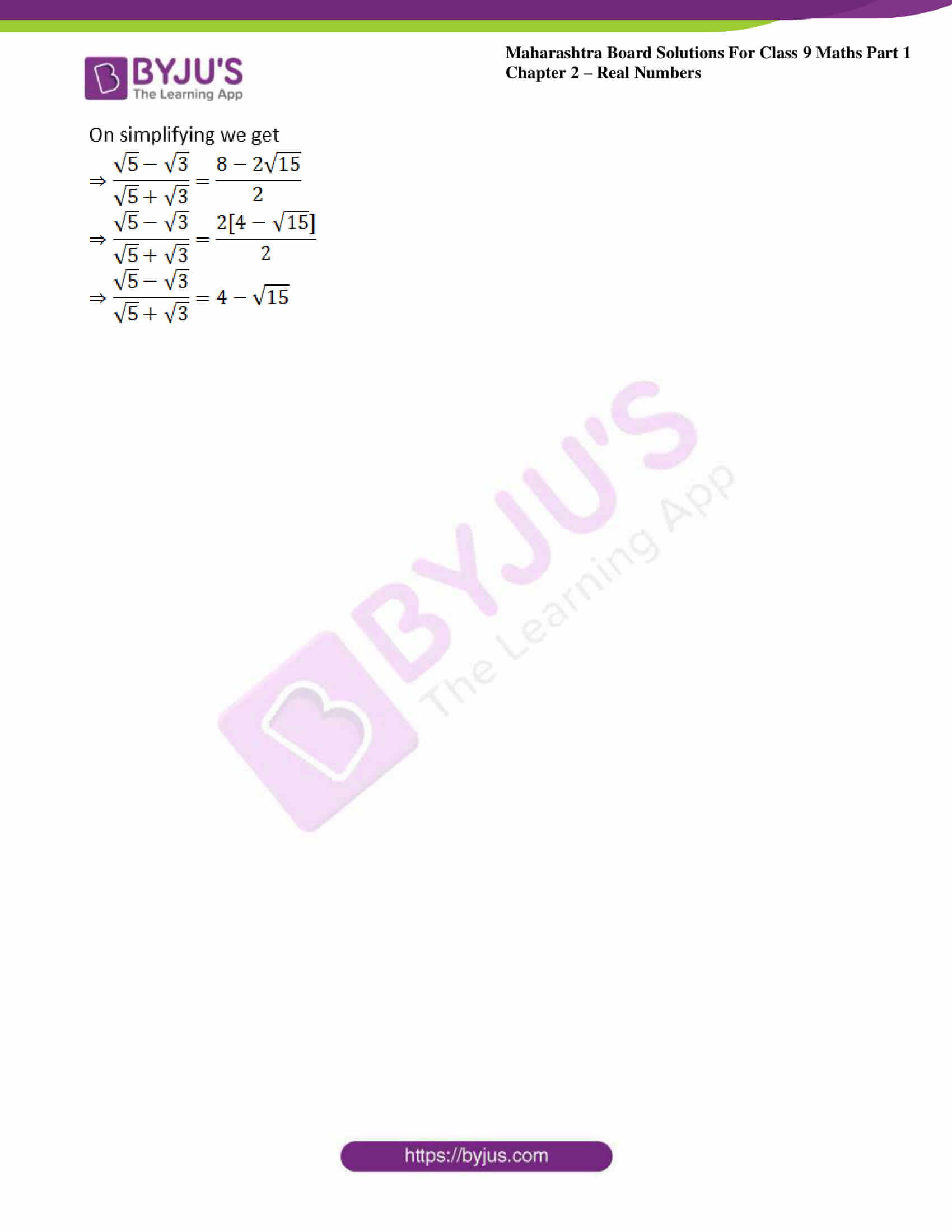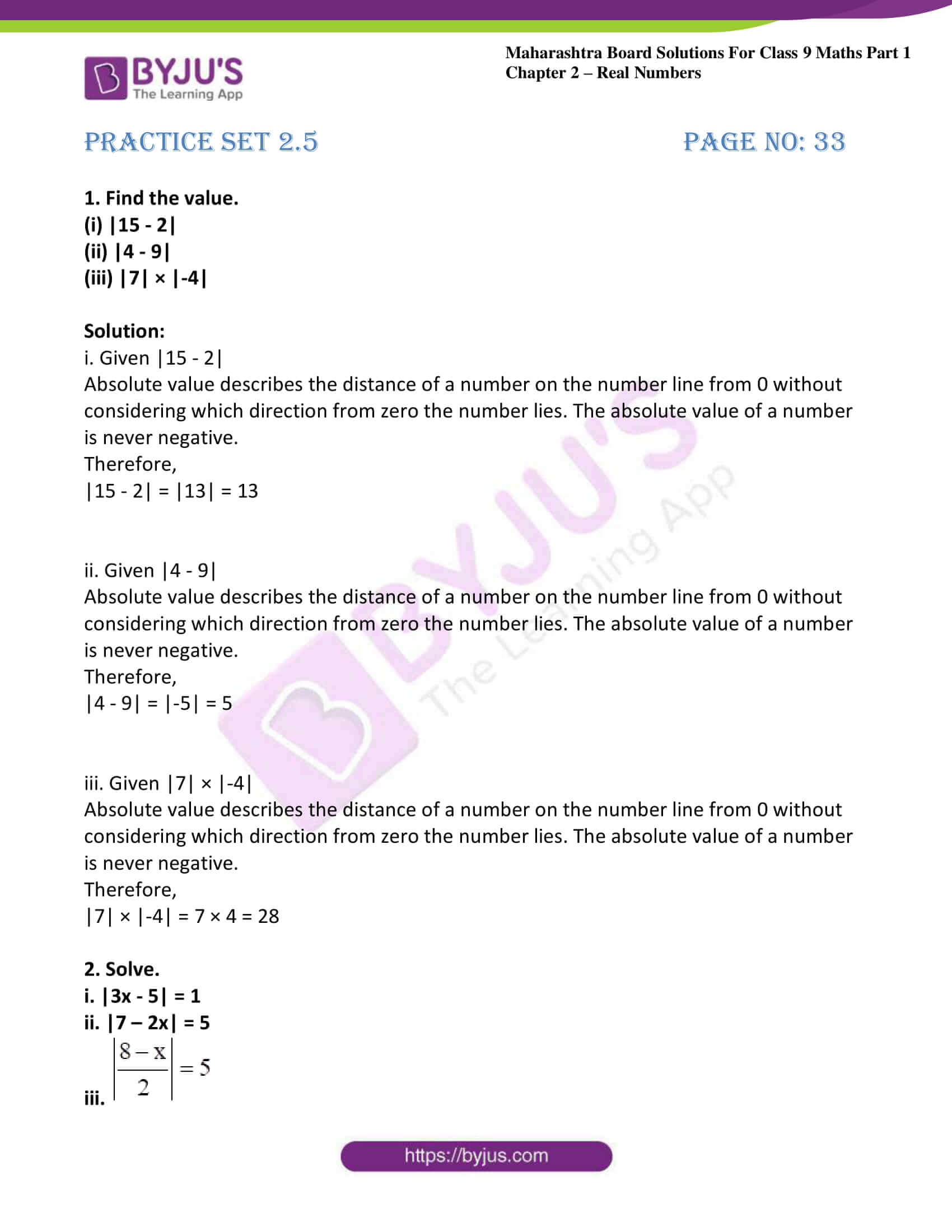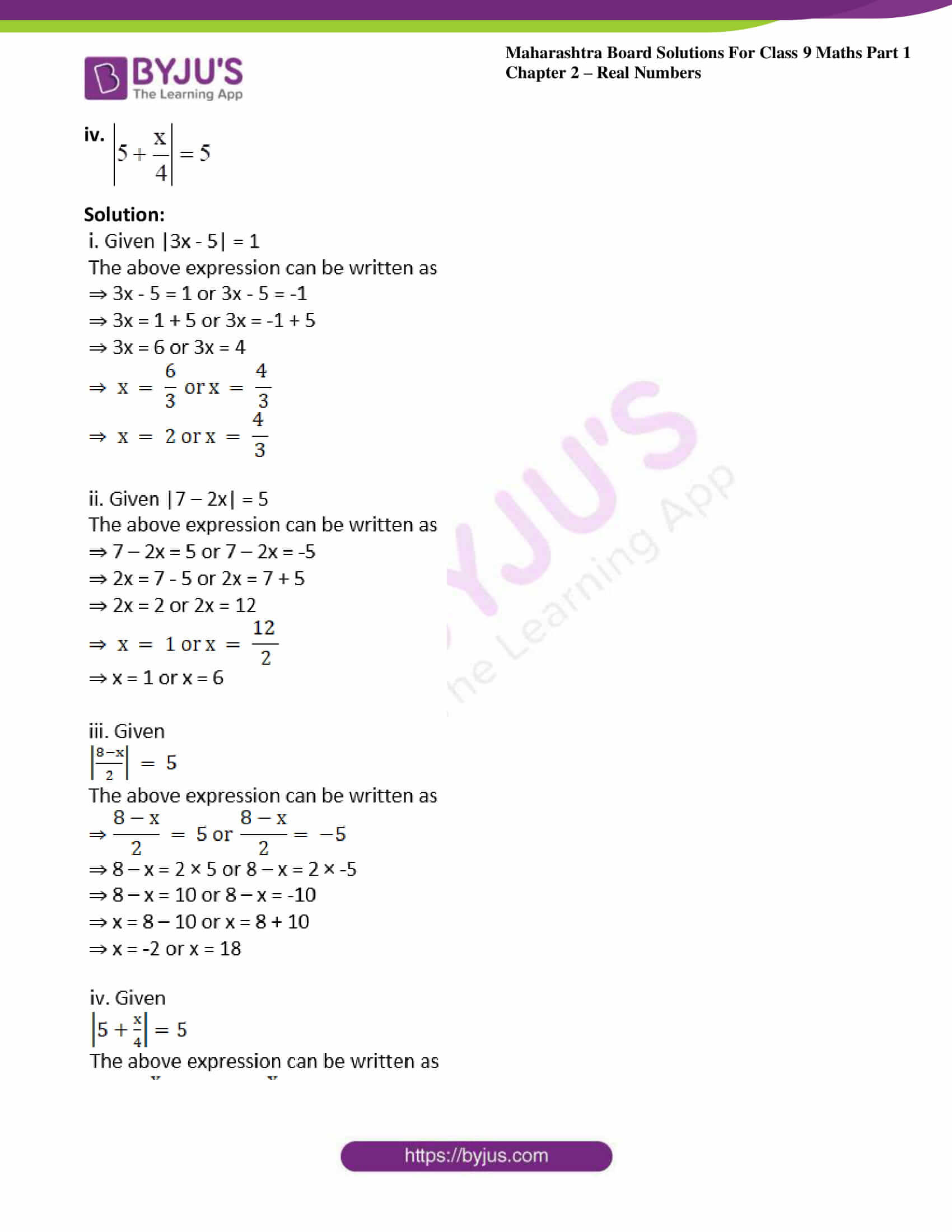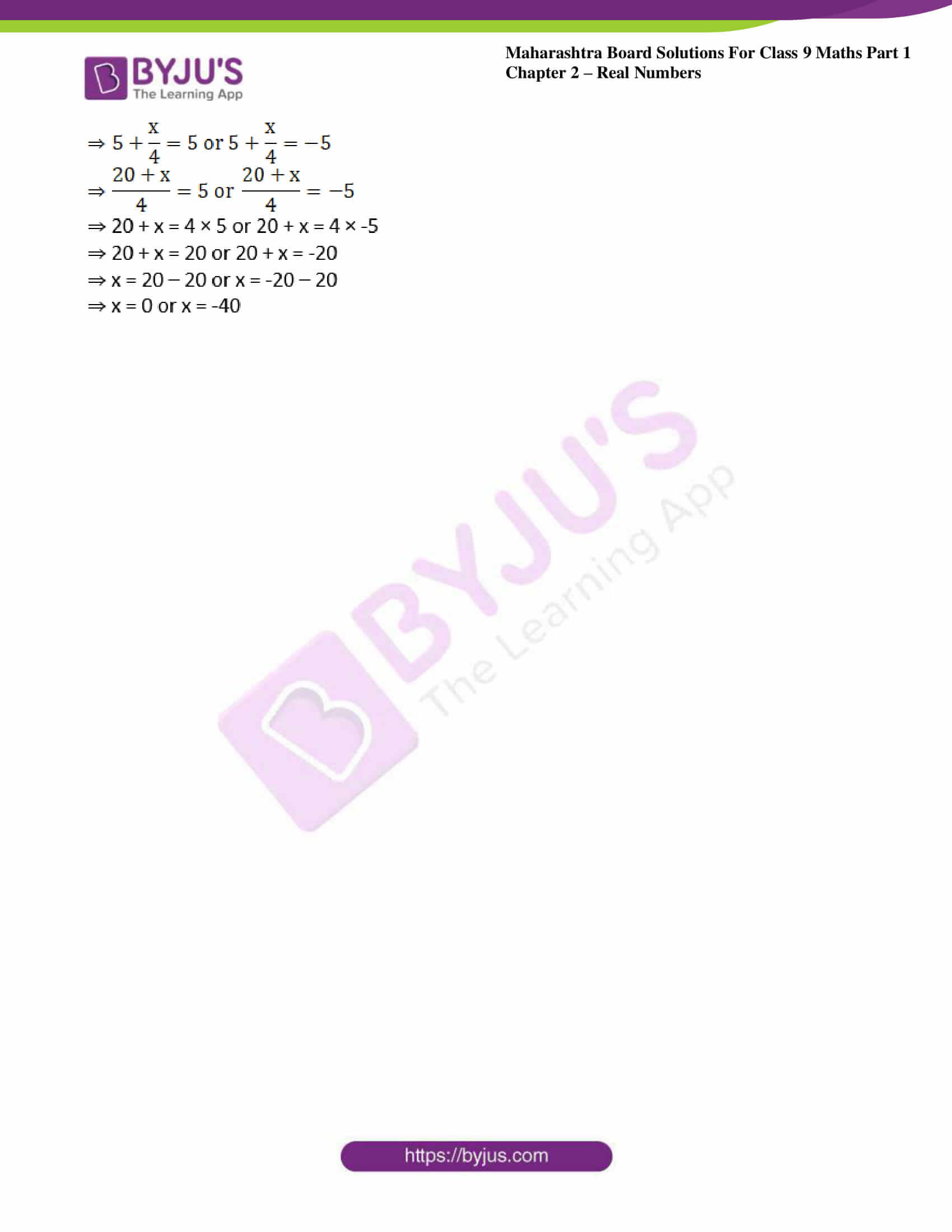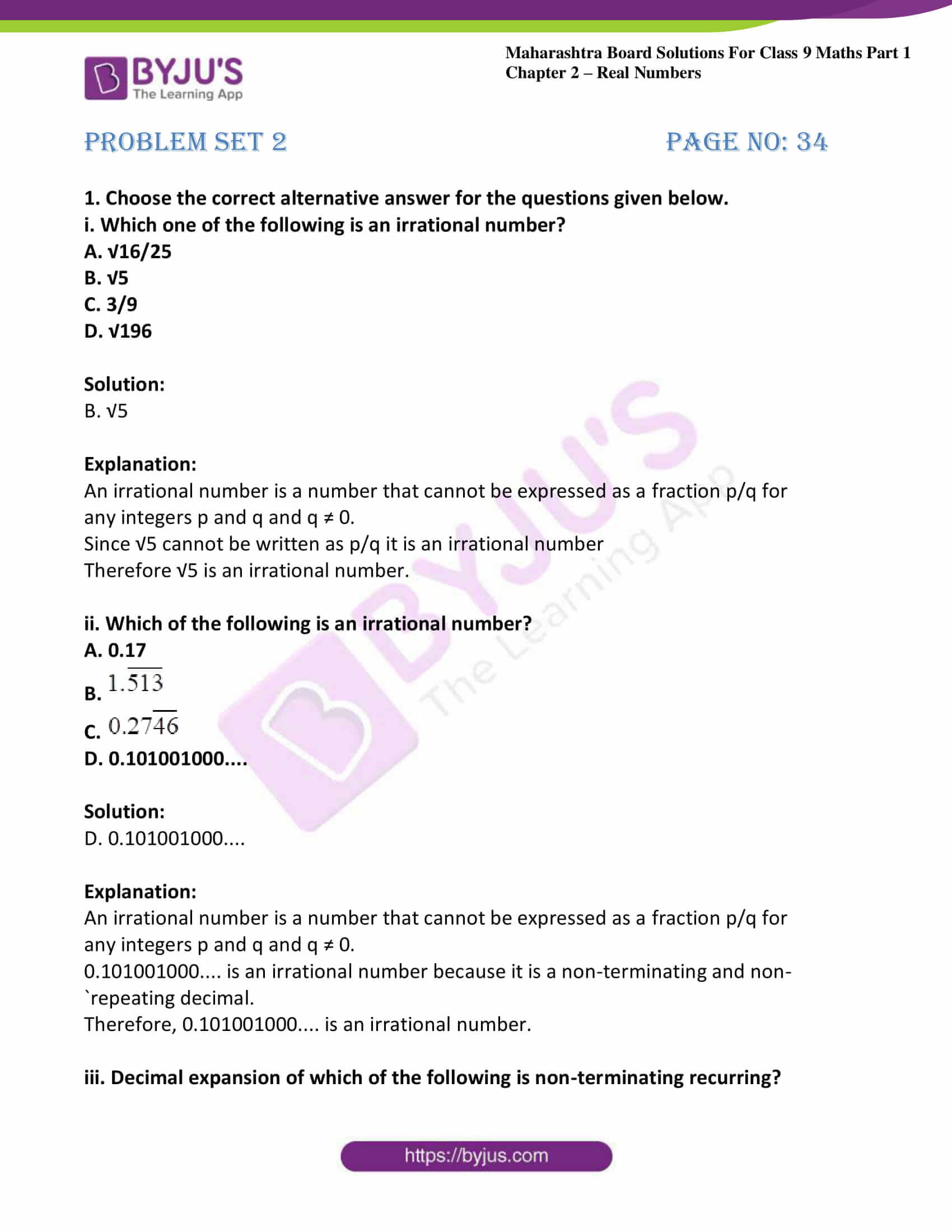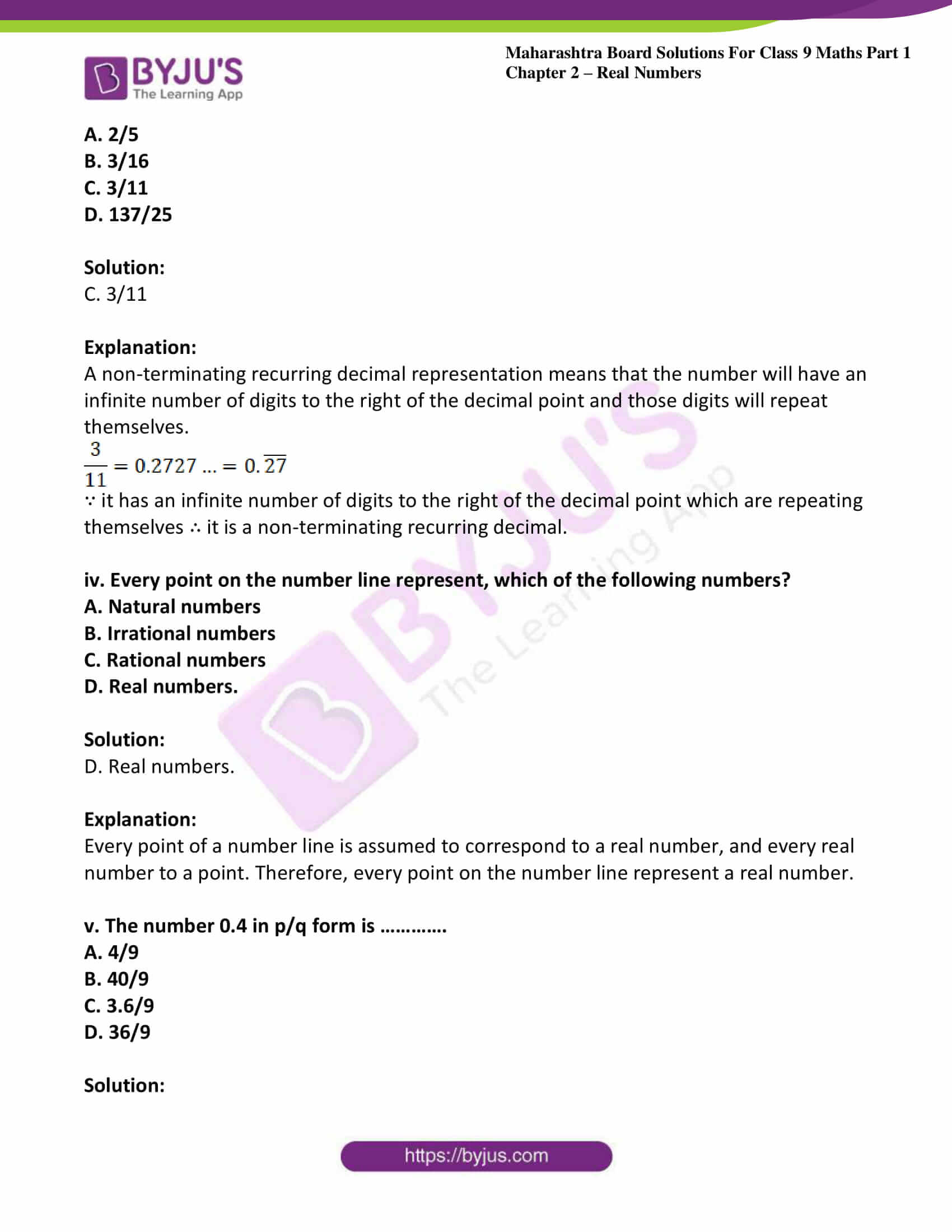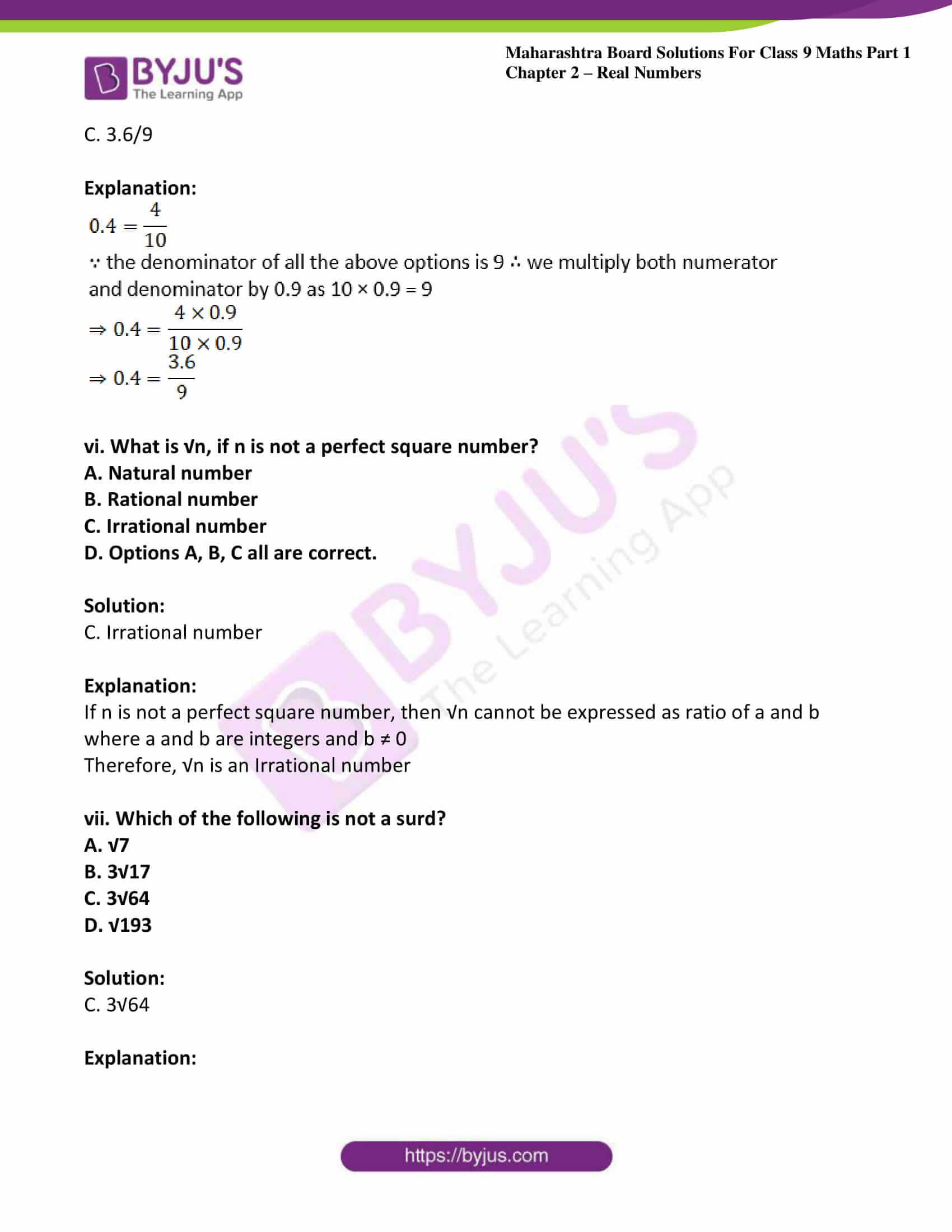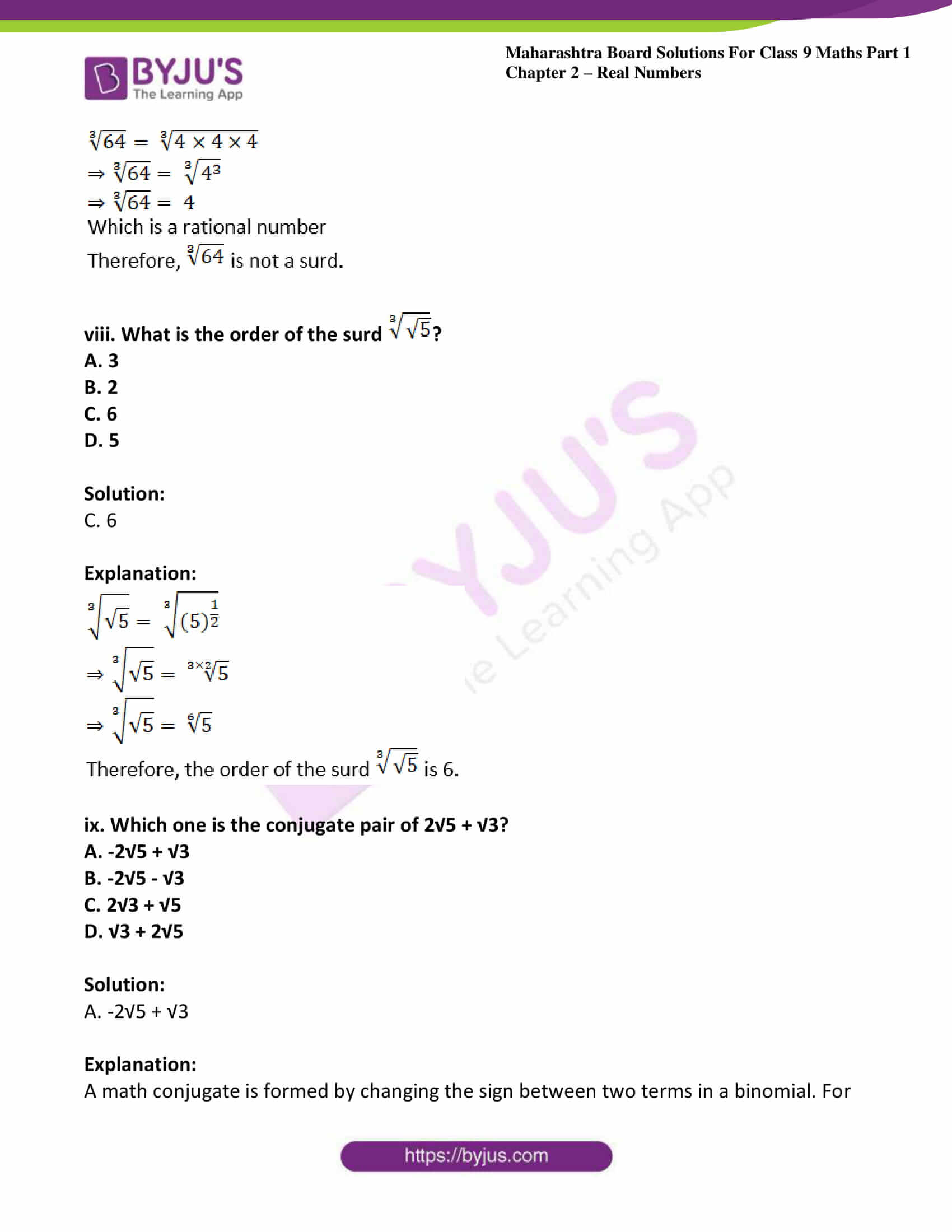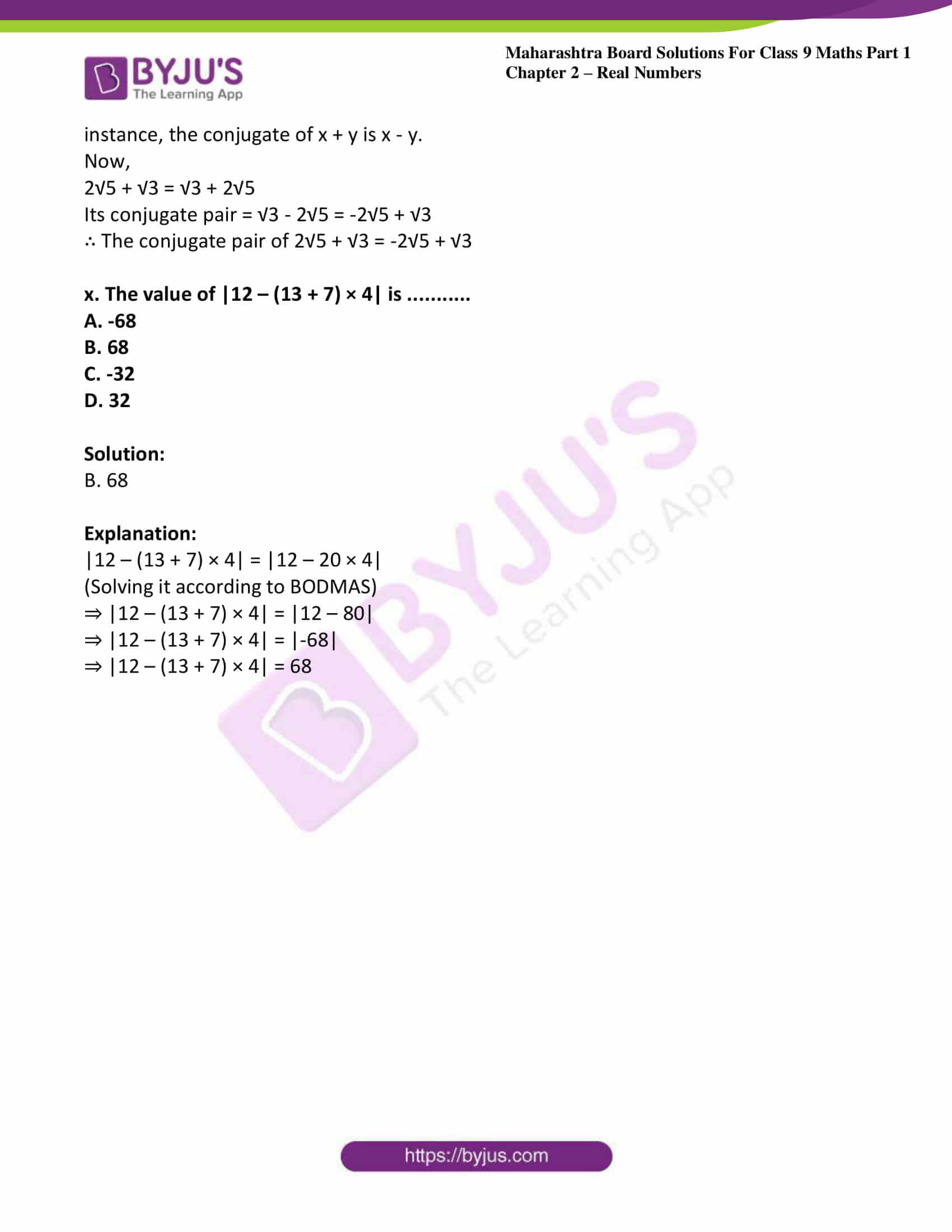### Access answers to Maths MSBSHSE Solutions For Class 9 Part 1 Chapter 2 – Real Numbers

Practice set 2.1 Page no: 21

1. Classify the decimal form of the given rational numbers into terminating and non-terminating recurring type.

i. 13/5

ii.
2/11
iii. 29/16

iv. 17/125
v. 11/6

Solution:

i.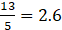∵ The division is exact

∴ it is a terminating decimal.

ii.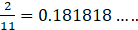∵ The division never ends and the digits ‘18’ is repeated endlessly

∴ it is a non-terminating recurring type decimal.

iii.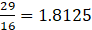∵ The division is exact

∴ it is a terminating decimal.

iv.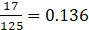∵ The division is exact

∴ it is a terminating decimal.

v.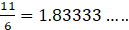∵ The division never ends and the digit ‘3’ is repeated endlessly

∴ it is a non-terminating recurring type decimal.

2. Write the following rational numbers in decimal form.

i. 127/200

ii. 25/99
iii. 23/7

iv. 4/5
v. 17/8

Solution:

i.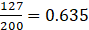ii.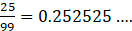iii.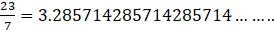iv.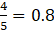v.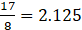3. Write the following rational numbers in form.
i.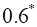ii.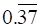iii.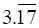iv.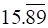v.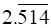Solution: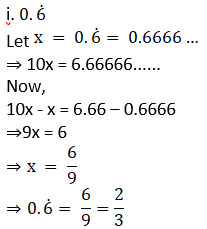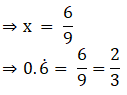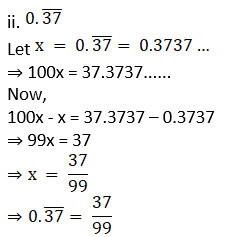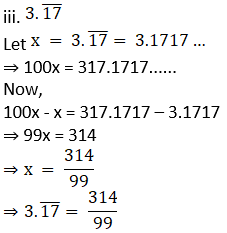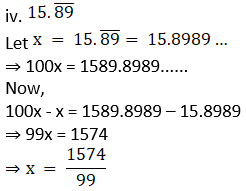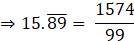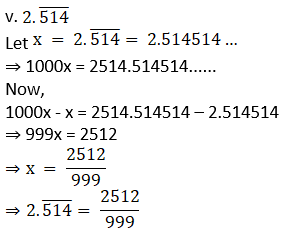Practice set 2.2 Page no: 25

1. Show that is 4√2 an irrational number.

Solution: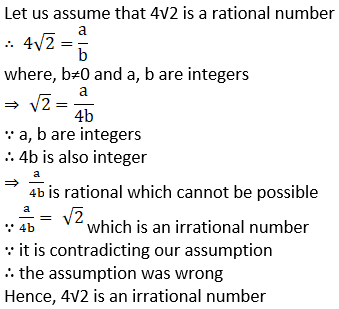2. Prove that 3 + √5 is an irrational number.

Solution: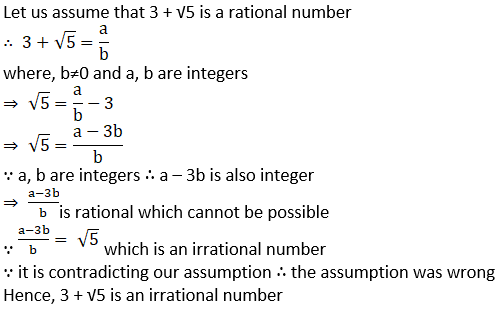3. Represent the numbers √5 and √10 on a number line.

Solution:

Given √5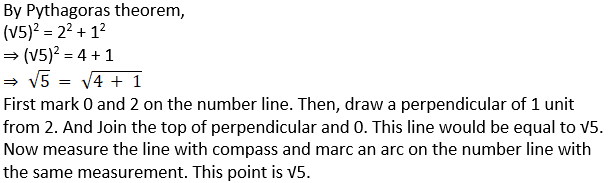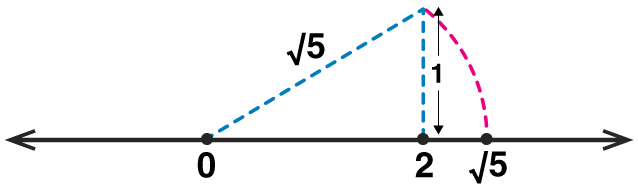Given that √10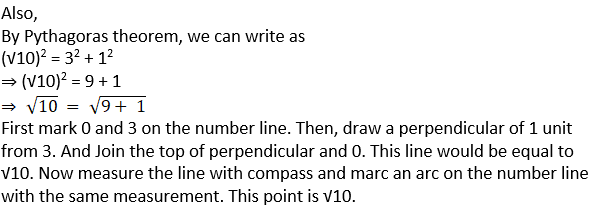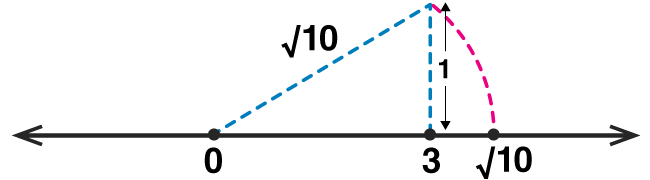4. Write any three rational numbers between the two numbers given below.

(i) 0.3 and -0.5

Solution: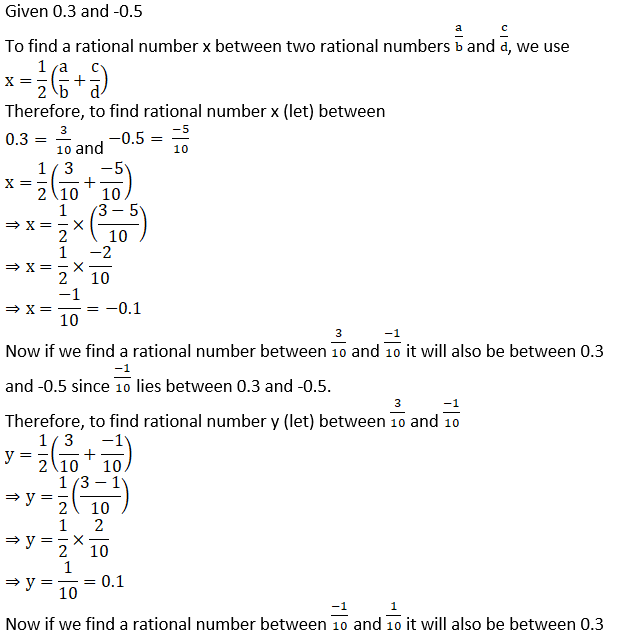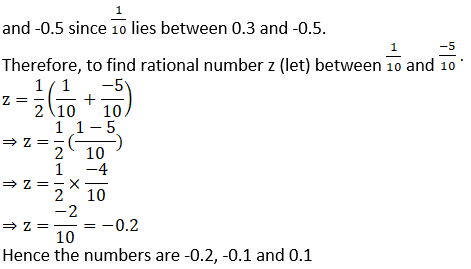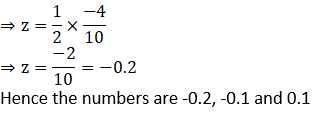(ii) -2.3 and -2.33

Solution: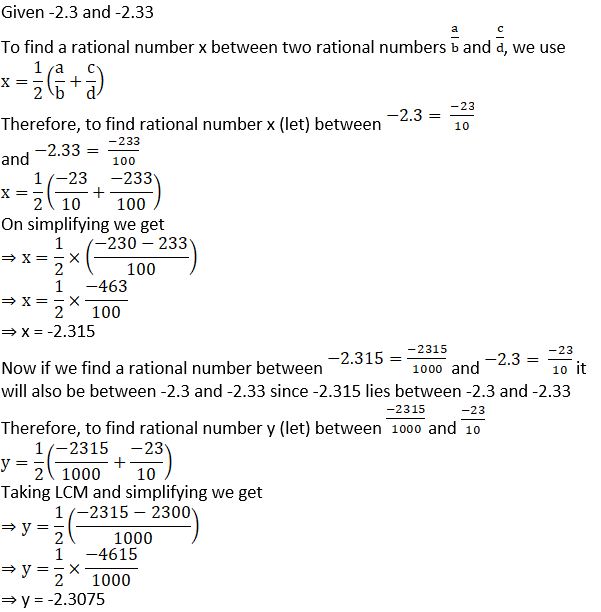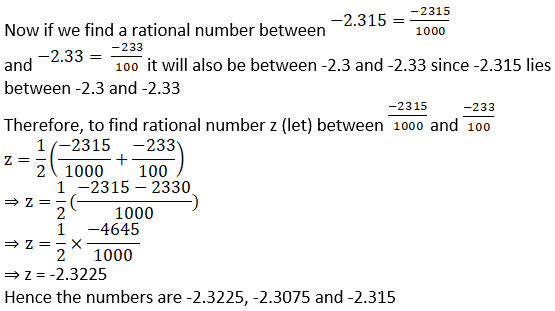(iii) 5.2 and 5.3

Solution: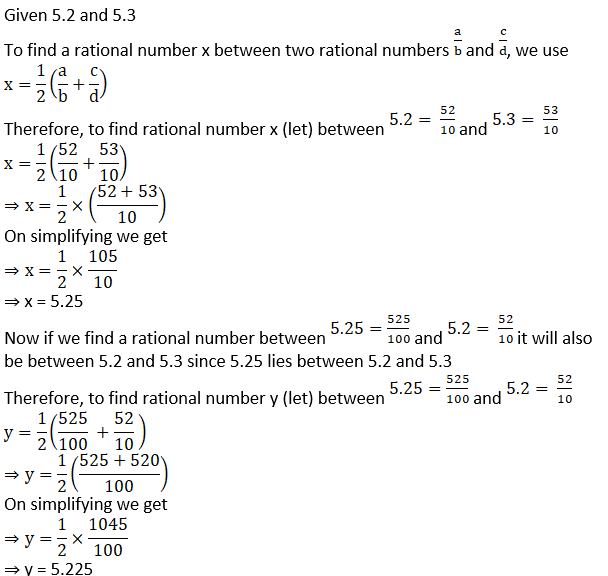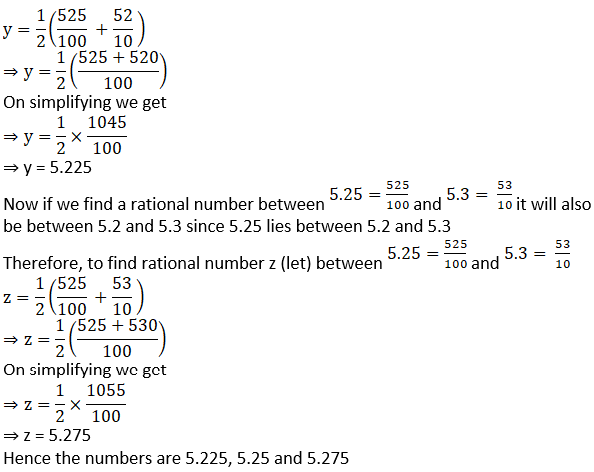(iv) -4.5 and 4.6

Solution: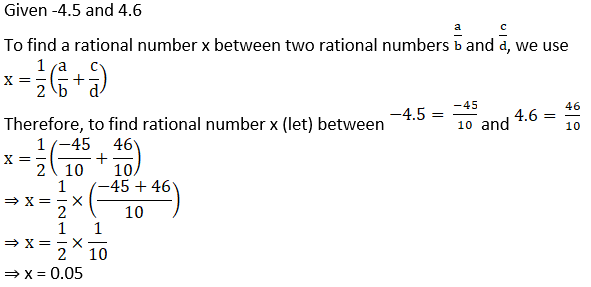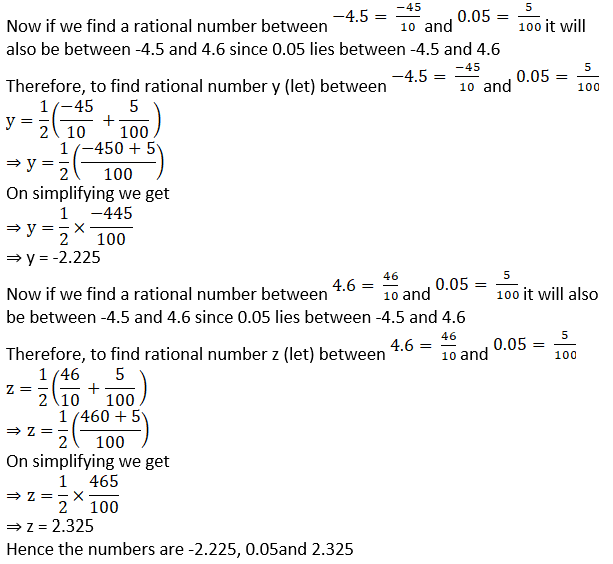Practice set 2.3 Page no: 30

1. State the order of the surds given below.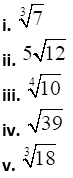Solution: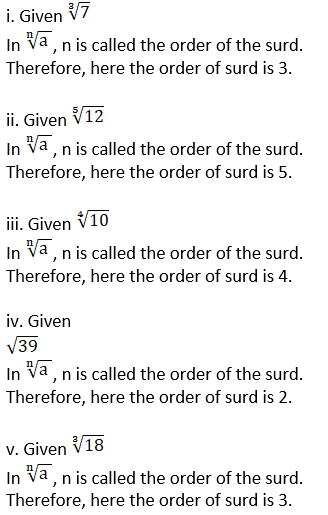2. State which of the following are surds. Justify.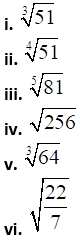Solution: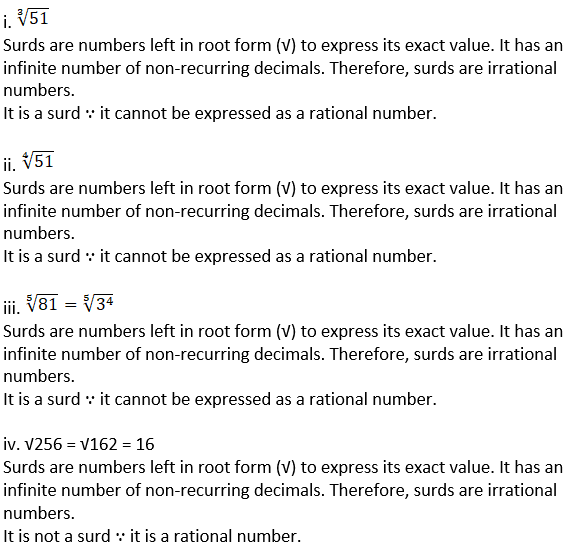iv. √256 = √162 = 16

Surds are numbers left in root form (√) to express its exact value. It has an infinite number of non-recurring decimals. Therefore, surds are irrational numbers.

It is not a surd ∵ it is a rational number.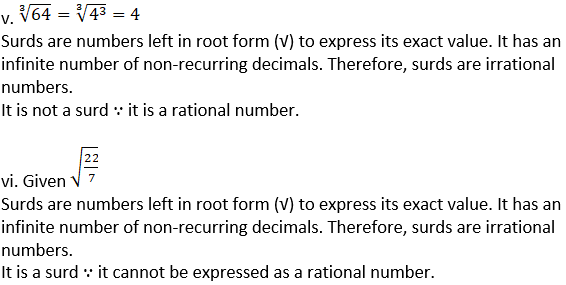3. Classify the given pair of surds into like surds and unlike surds.

i. √52, 5√13
ii. √68, 5√3
iii. 4√18, 7√2
iv. 19√12, 6√3
v. 5√22, 7√33
vi. 5√5, √75

Solution:

Two or more surds are said to be similar or like surds if they have the same surd-factor.

Two or more surds are said to be dissimilar or unlike when they are not similar.

Therefore,

i. Given √52, 5√13

given surd can be written as

√52 = √ (2×2×13) = 2√13

5√13

∵ both surds have same surd-factor that is √13.

∴ they are like surds.

ii. Given √68, 5√3

Given surd can be written as

√68 = √ (2×2×17) = 2√17

5√3

∵ both surds have different surd-factors √17 and √3.

∴ they are unlike surds.

iii. Given 4√18, 7√2

Given surd can be written as

4√18 = 4 √ (2×3×3) = 4×3√2 = 12√2

7√2

∵ both surds have same surd-factor i.e., √2.

∴ they are like surds.

iv. Given 19√12, 6√3

Given surd can be written as

19√12 = 19√ (2×2×3) = 19×2√3 = 38√3

6√3

∵ both surds have same surd-factor i.e., √3.

∴ they are like surds.

v. Given 5√22, 7√33

∵ both surds have different surd-factors √22 and √33.

∴ they are unlike surds.

vi. Given 5√5, √75

5√5

Given surd can be written as

√75 = √ (5×5×3) = 5√3

∵ both surds have different surd-factors √5 and √3.

∴ they are unlike surds.

4. Simplify the following surds.
i. √27
ii. √50
iii. √250
iv. √112
v. √168

Solution: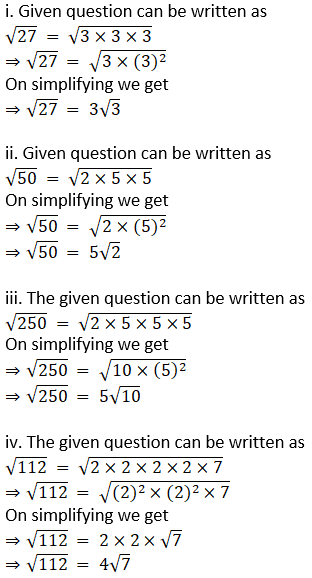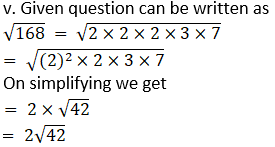Practice set 2.4 Page no: 32

1. Multiply
i. √3(√7 – √3)
ii. (√5 – √7) √2
iii. (3√2 – √3) (4√3 – √2)

Solution:

i. Given √3 (√7 – √3)

=√3 × √7 – √3 × √3

[∵ √a (√b – √c) = √a × √b – √a × √c]

=√21 – 3

ii. Given (√5 – √7) √2

=√5 × √2 – √7 × √2

[∵√a (√b – √c) = √a × √b – √a × √c]

= √10 – √14

iii. Given (3√2 – √3) (4√3 – √2)

=3√2 (4√3 – √2) – √3 (4√3 – √2)

[∵ √a (√b – √c) = √a × √b – √a × √c]

= 3√2 × 4√3 – 3√2 × √2 – √3 × 4√3 + √3 × √2

= 12√6 – 3 × 2 – 4 × 3 + √6

= 12√6 – 6 – 12 + √6

= 13√6 – 18

2. Rationalize the denominator.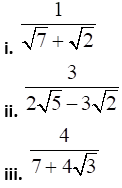iv.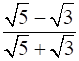Solution: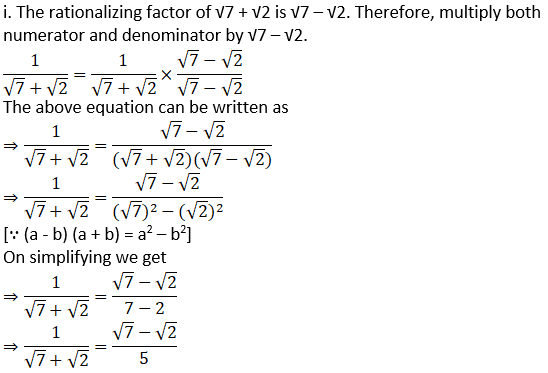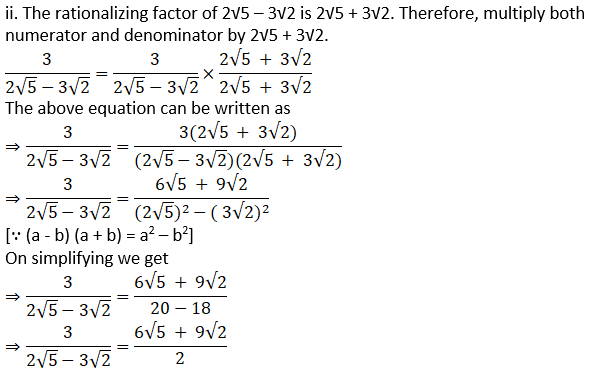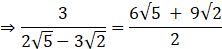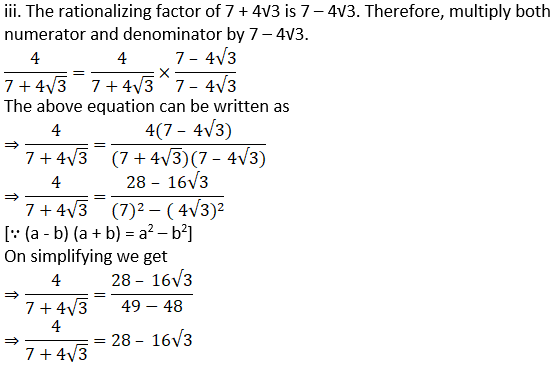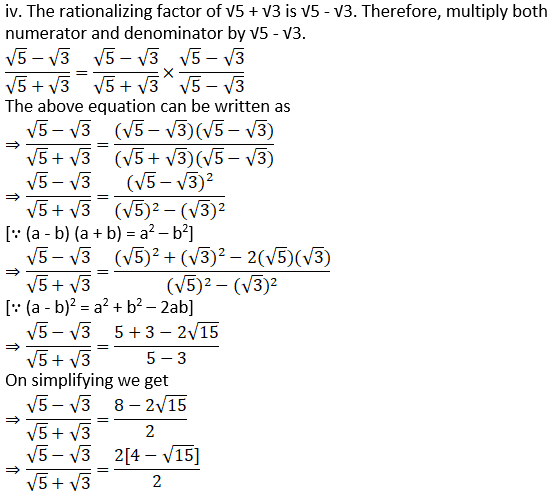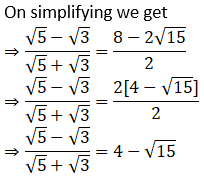Practice set 2.5 Page no: 33

1. Find the value.
(i) |15 – 2|
(ii) |4 – 9|
(iii) |7| × |-4|

Solution:

i. Given |15 – 2|

Absolute value describes the distance of a number on the number line from 0 without considering which direction from zero the number lies. The absolute value of a number is never negative.

Therefore,

|15 – 2| = |13| = 13

ii. Given |4 – 9|

Absolute value describes the distance of a number on the number line from 0 without considering which direction from zero the number lies. The absolute value of a number is never negative.

Therefore,

|4 – 9| = |-5| = 5

iii. Given |7| × |-4|

Absolute value describes the distance of a number on the number line from 0 without considering which direction from zero the number lies. The absolute value of a number is never negative.

Therefore,

|7| × |-4| = 7 × 4 = 28

2. Solve.
i. |3x – 5| = 1
ii. |7 – 2x| = 5
iii.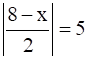iv.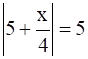Solution: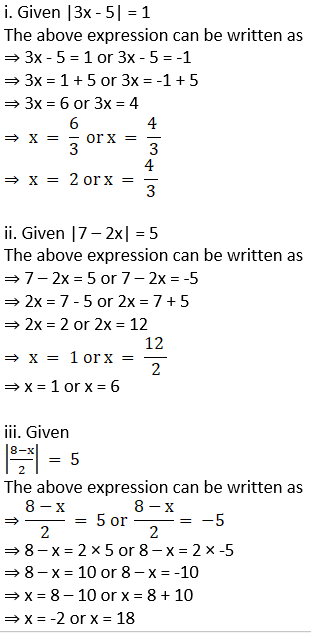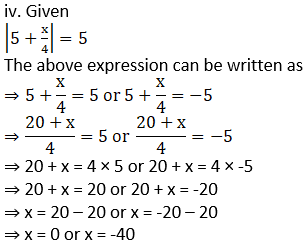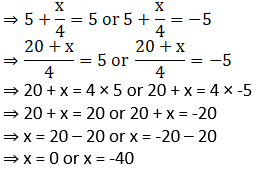Problem set 2 Page no: 34

1. Choose the correct alternative answer for the questions given below.
i. Which one of the following is an irrational number?
A. √16/25
B. √5
C. 3/9
D. √196

Solution:

B. √5

Explanation:

An irrational number is a number that cannot be expressed as a fraction p/q for any integers p and q and q ≠ 0.

Since √5 cannot be written as p/q it is an irrational number

Therefore √5 is an irrational number.

ii. Which of the following is an irrational number?
A. 0.17
B.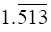C.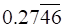D. 0.101001000….

Solution:

D. 0.101001000….

Explanation:

An irrational number is a number that cannot be expressed as a fraction p/q for any integers p and q and q ≠ 0.

0.101001000…. is an irrational number because it is a non-terminating and non-`repeating decimal.

Therefore, 0.101001000…. is an irrational number.

iii. Decimal expansion of which of the following is non-terminating recurring?
A. 2/5
B. 3/16
C. 3/11
D. 137/25

Solution:

C. 3/11

Explanation:

A non-terminating recurring decimal representation means that the number will have an infinite number of digits to the right of the decimal point and those digits will repeat themselves.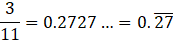∵ it has an infinite number of digits to the right of the decimal point which are repeating themselves ∴ it is a non-terminating recurring decimal.

iv. Every point on the number line represent, which of the following numbers?
A. Natural numbers
B. Irrational numbers
C. Rational numbers
D. Real numbers.

Solution:

D. Real numbers.

Explanation:

Every point of a number line is assumed to correspond to a real number, and every real number to a point. Therefore, every point on the number line represent a real number.

v. The number 0.4 in p/q form is ………….
A. 4/9
B. 40/9
C. 3.6/9
D. 36/9

Solution:

C. 3.6/9

Explanation: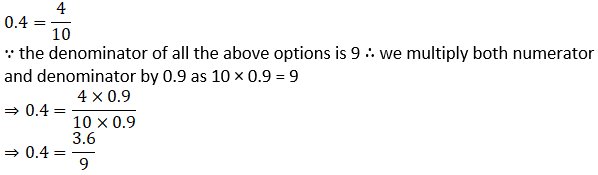vi. What is √n, if n is not a perfect square number?
A. Natural number
B. Rational number
C. Irrational number
D. Options A, B, C all are correct.

Solution:

C. Irrational number

Explanation:

If n is not a perfect square number, then √n cannot be expressed as ratio of a and b where a and b are integers and b ≠ 0

Therefore, √n is an Irrational number

vii. Which of the following is not a surd?
A. √7
B. 3√17
C. 3√64
D. √193

Solution:

C. 3√64

Explanation: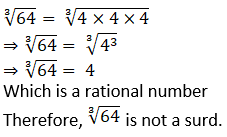viii. What is the order of the surd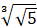?
A. 3
B. 2
C. 6
D. 5

Solution:

C. 6

Explanation: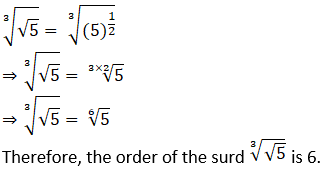ix. Which one is the conjugate pair of 2√5 + √3?
A. -2√5 + √3
B. -2√5 – √3
C. 2√3 + √5
D. √3 + 2√5

Solution:

A. -2√5 + √3

Explanation:

A math conjugate is formed by changing the sign between two terms in a binomial. For instance, the conjugate of x + y is x – y.

Now,

2√5 + √3 = √3 + 2√5

Its conjugate pair = √3 – 2√5 = -2√5 + √3

∴ The conjugate pair of 2√5 + √3 = -2√5 + √3

x. The value of |12 – (13 + 7) × 4| is ………..
A. -68
B. 68
C. -32
D. 32

Solution:

B. 68

Explanation:

|12 – (13 + 7) × 4| = |12 – 20 × 4|

(Solving it according to BODMAS)

⇒ |12 – (13 + 7) × 4| = |12 – 80|

⇒ |12 – (13 + 7) × 4| = |-68|

⇒ |12 – (13 + 7) × 4| = 68

Real numbers are simply the combination of rational and irrational numbers, in the number system. In general, all the arithmetic operations can be performed on these numbers and they can be represented in the number line. Students can depend on these Solutions to understand all the topics completely. Stay tuned to learn more about Real Numbers, MSBSHSE Exam pattern and other information.

## Frequently Asked Questions on Maharashtra State Board Solutions for Class 9 Maths Part 1 Chapter 2 Real Numbers

### Will I have to make any payment to access these Maharashtra State Board Solutions for Class 9 Maths Part 1 Chapter 2 Real Numbers?

No, there is no need to pay any money. We have made these solutions available online free for download. Students can access them from our site by entering the login details. Or else, the scrollable PDF is also accessible. Those who wish to can also check out the questions and the solutions from our webpage.

### Are these Maharashtra State Board Solutions for Class 9 Maths Part 1 Chapter 2 Real Numbers helpful?

Yes. These solutions are useful and they set the basis for questions that could get asked in the board exams. Students are also advised to practise these questions first and then refer back to the solutions to analyse their performance and then rectify the mistakes, so that they can avoid making any during the board exams.

### Is Maharashtra Board Class 9 Maths Solutions of Chapter 2 difficult?

No, Maharashtra Board Class 9 Maths Solutions of Chapter 2  Real Numbers is not half as difficult as it seems. You can easily understand concepts of the chapter if you refer to this chapterwise solutions . You can also go through your syllabus course structure and unit-wise weightage thoroughly to know how much weightage the chapter will carry for the final exam.